• 最短路径问题是图论研究的一个经典算法问题，旨在寻找（由结点和路径组成的）两结点之间的最短路径。 算法具体的形式包括： 确定起点的最短路径问题：即已知起始结点，求最短路径的问题。适合使用Dijkstra...前言
本专题旨在快速了解常见的数据结构和算法。
在需要使用到相应算法时，能够帮助你回忆出常用的实现方案并且知晓其优缺点和适用环境。并不涉及十分具体的实现细节描述。
图的最短路径算法
最短路径问题是图论研究中的一个经典算法问题，旨在寻找图（由结点和路径组成的）中两结点之间的最短路径。
算法具体的形式包括：
确定起点的最短路径问题：即已知起始结点，求最短路径的问题。适合使用Dijkstra算法。确定终点的最短路径问题：与确定起点的问题相反，该问题是已知终结结点，求最短路径的问题。在无向图中该问题与确定起点的问题完全等同，在有向图中该问题等同于把所有路径方向反转的确定起点的问题。确定起点终点的最短路径问题：即已知起点和终点，求两结点之间的最短路径。全局最短路径问题：求图中所有的最短路径。适合使用Floyd-Warshall算法。
主要介绍以下几种算法：
Dijkstra最短路算法（单源最短路）Bellman–Ford算法（解决负权边问题）SPFA算法（Bellman-Ford算法改进版本）Floyd最短路算法（全局/多源最短路）
常用算法
Dijkstra最短路算法（单源最短路）
图片例子和史料来自：http://blog.51cto.com/ahalei/1387799
算法介绍：
迪科斯彻算法使用了广度优先搜索解决赋权有向图或者无向图的单源最短路径问题，算法最终得到一个最短路径树。该算法常用于路由算法或者作为其他图算法的一个子模块。
指定一个起始点（源点）到其余各个顶点的最短路径，也叫做“单源最短路径”。例如求下图中的1号顶点到2、3、4、5、6号顶点的最短路径。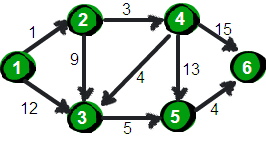使用二维数组e来存储顶点之间边的关系，初始值如下。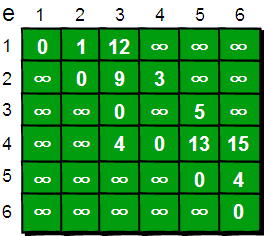我们还需要用一个一维数组dis来存储1号顶点到其余各个顶点的初始路程，如下。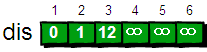将此时dis数组中的值称为最短路的“估计值”。
既然是求1号顶点到其余各个顶点的最短路程，那就先找一个离1号顶点最近的顶点。通过数组dis可知当前离1号顶点最近是2号顶点。当选择了2号顶点后，dis的值就已经从“估计值”变为了“确定值”，即1号顶点到2号顶点的最短路程就是当前dis值。
既然选了2号顶点，接下来再来看2号顶点有哪些出边呢。有2->3和2->4这两条边。先讨论通过2->3这条边能否让1号顶点到3号顶点的路程变短。也就是说现在来比较dis和dis+e的大小。其中dis表示1号顶点到3号顶点的路程。dis+e中dis表示1号顶点到2号顶点的路程，e表示2->3这条边。所以dis+e就表示从1号顶点先到2号顶点，再通过2->3这条边，到达3号顶点的路程。
这个过程有个专业术语叫做“松弛”。松弛完毕之后dis数组为：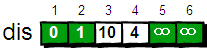接下来，继续在剩下的3、4、5和6号顶点中，选出离1号顶点最近的顶点4，变为确定值，以此类推。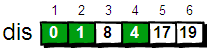最终dis数组如下，这便是1号顶点到其余各个顶点的最短路径。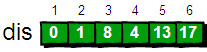核心代码：
//Dijkstra算法核心语句
for(i=1;i<=n-1;i++)
{
//找到离1号顶点最近的顶点
min=inf;
for(j=1;j<=n;j++)
{
if(book[j]==0 && dis[j]<min)
{
min=dis[j];
u=j;
}
}
book[u]=1;
for(v=1;v<=n;v++)
{
if(e[u][v]<inf)
{
if(dis[v]>dis[u]+e[u][v])
dis[v]=dis[u]+e[u][v];
}
}
}

关于复杂度：
M：边的数量N：节点数量
通过上面的代码我们可以看出，我们实现的Dijkstra最短路算法的时间复杂度是O(N^2)。其中每次找到离1号顶点最近的顶点的时间复杂度是O(N)。
优化:
这里我们可以用“堆”（以后再说）来优化，使得这一部分的时间复杂度降低到O(logN)。  另外对于边数M少于N^2的稀疏图来说（我们把M远小于N^2的图称为稀疏图，而M相对较大的图称为稠密图），我们可以用邻接表来代替邻接矩阵，使得整个时间复杂度优化到O((M+N)logN)。  请注意！在最坏的情况下M就是N^2，这样的话MlogN要比N^2还要大。但是大多数情况下并不会有那么多边，因此(M+N)logN要比N^2小很多。
Dijkstra思想总结：
dijkstra算法本质上算是贪心的思想，每次在剩余节点中找到离起点最近的节点放到队列中，并用来更新剩下的节点的距离，再将它标记上表示已经找到到它的最短路径，以后不用更新它了。这样做的原因是到一个节点的最短路径必然会经过比它离起点更近的节点，而如果一个节点的当前距离值比任何剩余节点都小，那么当前的距离值一定是最小的。（剩余节点的距离值只能用当前剩余节点来更新，因为求出了最短路的节点之前已经更新过了）
dijkstra就是这样不断从剩余节点中拿出一个可以确定最短路径的节点最终求得从起点到每个节点的最短距离。
用邻接表代替邻接矩阵存储
参考：http://blog.51cto.com/ahalei/1391988
总结如下：
可以发现使用邻接表来存储图的时间空间复杂度是O(M)，遍历每一条边的时间复杂度是也是O(M)。如果一个图是稀疏图的话，M要远小于N^2。因此稀疏图选用邻接表来存储要比邻接矩阵来存储要好很多。
Bellman–Ford算法（解决负权边问题）
思想：
bellman-ford算法进行n-1次更新（一次更新是指用所有节点进行一次松弛操作）来找到到所有节点的单源最短路。
bellman-ford算法和dijkstra其实有点相似，该算法能够保证每更新一次都能确定一个节点的最短路，但与dijkstra不同的是，并不知道是那个节点的最短路被确定了，只是知道比上次多确定一个，这样进行n-1次更新后所有节点的最短路都确定了（源点的距离本来就是确定的）。
现在来说明为什么每次更新都能多找到一个能确定最短路的节点：
1.将所有节点分为两类：已知最短距离的节点和剩余节点。

2.这两类节点满足这样的性质：已知最短距离的节点的最短距离值都比剩余节点的最短路值小。（这一点也和dijkstra一样）

3.有了上面两点说明，易知到剩余节点的路径一定会经过已知节点

4.而从已知节点连到剩余节点的所有边中的最小的那个边，这条边所更新后的剩余节点就一定是确定的最短距离，从而就多找到了一个能确定最短距离的节点，不用知道它到底是哪个节点。


bellman-ford的一个优势是可以用来判断是否存在负环，在不存在负环的情况下，进行了n-1次所有边的更新操作后每个节点的最短距离都确定了，再用所有边去更新一次不会改变结果。而如果存在负环，最后再更新一次会改变结果。原因是之前是假定了起点的最短距离是确定的并且是最短的，而又负环的情况下这个假设不再成立。

Bellman-Ford 算法描述：
创建源顶点 v 到图中所有顶点的距离的集合 distSet，为图中的所有顶点指定一个距离值，初始均为 Infinite，源顶点距离为 0；计算最短路径，执行 V - 1 次遍历；
对于图中的每条边：如果起点 u 的距离 d 加上边的权值 w 小于终点 v 的距离 d，则更新终点 v 的距离值 d； 检测图中是否有负权边形成了环，遍历图中的所有边，计算 u 至 v 的距离，如果对于 v 存在更小的距离，则说明存在环；
伪代码：
BELLMAN-FORD(G, w, s)
INITIALIZE-SINGLE-SOURCE(G, s)
for i  1 to |V[G]| - 1
do for each edge (u, v)  E[G]
do RELAX(u, v, w)
for each edge (u, v)  E[G]
do if d[v] > d[u] + w(u, v)
then return FALSE
return TRUE

SPFA（Bellman-Ford算法改进版本）

SPFA算法是1994年西安交通大学段凡丁提出

spfa可以看成是bellman-ford的队列优化版本，正如在前面讲到的，bellman每一轮用所有边来进行松弛操作可以多确定一个点的最短路径，但是用每次都把所有边拿来松弛太浪费了，不难发现，只有那些已经确定了最短路径的点所连出去的边才是有效的，因为新确定的点一定要先通过已知(最短路径的)节点。
所以我们只需要把已知节点连出去的边用来松弛就行了，但是问题是我们并不知道哪些点是已知节点，不过我们可以放宽一下条件，找哪些可能是已知节点的点，也就是之前松弛后更新的点，已知节点必然在这些点中。 所以spfa的做法就是把每次更新了的点放到队列中记录下来。
伪代码：
ProcedureSPFA;
Begin
initialize-single-source(G,s);
initialize-queue(Q);
enqueue(Q,s);
while not empty(Q) do begin
u:=dequeue(Q);
tmp:=d[v];
relax(u,v);
if(tmp<>d[v])and(not v in Q)then enqueue(Q,v);
end;
end;
End;

如何看待 SPFA 算法已死这种说法？
在非负边权的图中，随手卡 SPFA 已是业界常识。在负边权的图中，不把 SPFA 卡到最慢就设定时限是非常不负责任的行为，而卡到最慢就意味着 SPFA 和传统 Bellman Ford 算法的时间效率类似，而后者的实现难度远低于前者。
Floyd最短路算法（全局/多源最短路）
图片例子和史料来自：https://www.cnblogs.com/ahalei/p/3622328.html

此算法由Robert W. Floyd（罗伯特·弗洛伊德）于1962年发表在“Communications of the ACM”上。同年Stephen Warshall（史蒂芬·沃舍尔）也独立发表了这个算法。Robert W．Floyd这个牛人是朵奇葩，他原本在芝加哥大学读的文学，但是因为当时美国经济不太景气，找工作比较困难，无奈之下到西屋电气公司当了一名计算机操作员，在IBM650机房值夜班，并由此开始了他的计算机生涯。此外他还和J.W.J. Williams（威廉姆斯）于1964年共同发明了著名的堆排序算法HEAPSORT。

算法介绍：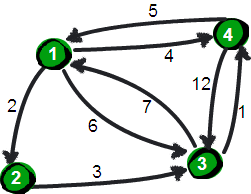上图中有4个城市8条公路，公路上的数字表示这条公路的长短。请注意这些公路是单向的。我们现在需要求任意两个城市之间的最短路程，也就是求任意两个点之间的最短路径。这个问题这也被称为“多源最短路径”问题。
现在需要一个数据结构来存储图的信息，我们仍然可以用一个4*4的矩阵（二维数组e）来存储。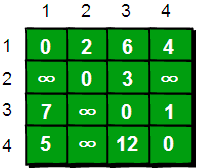核心代码：
for(k=1;k<=n;k++)
for(i=1;i<=n;i++)
for(j=1;j<=n;j++)
if(e[i][j]>e[i][k]+e[k][j])
e[i][j]=e[i][k]+e[k][j];

这段代码的基本思想就是：
最开始只允许经过1号顶点进行中转，接下来只允许经过1和2号顶点进行中转……允许经过1~n号所有顶点进行中转，求任意两点之间的最短路程。一旦发现比之前矩阵内存储的距离短，就用它覆盖原来保存的距离。
用一句话概括就是：从i号顶点到j号顶点只经过前k号点的最短路程。

另外需要注意的是：Floyd-Warshall算法不能解决带有“负权回路”（或者叫“负权环”）的图，因为带有“负权回路”的图没有最短路。例如下面这个图就不存在1号顶点到3号顶点的最短路径。因为1->2->3->1->2->3->…->1->2->3这样路径中，每绕一次1->-2>3这样的环，最短路就会减少1，永远找不到最短路。其实如果一个图中带有“负权回路”那么这个图则没有最短路。

代码实现：
#include <stdio.h>
int main()
{
int e,k,i,j,n,m,t1,t2,t3;
int inf=99999999; //用inf(infinity的缩写)存储一个我们认为的正无穷值
//读入n和m，n表示顶点个数，m表示边的条数
scanf("%d %d",&n,&m);

//初始化
for(i=1;i<=n;i++)
for(j=1;j<=n;j++)
if(i==j) e[i][j]=0;
else e[i][j]=inf;

//读入边
for(i=1;i<=m;i++)
{
scanf("%d %d %d",&t1,&t2,&t3);
e[t1][t2]=t3;
}

//Floyd-Warshall算法核心语句
for(k=1;k<=n;k++)
for(i=1;i<=n;i++)
for(j=1;j<=n;j++)
if(e[i][j]>e[i][k]+e[k][j] )
e[i][j]=e[i][k]+e[k][j];

//输出最终的结果
for(i=1;i<=n;i++)
{
for(j=1;j<=n;j++)
{
printf("%10d",e[i][j]);
}
printf("\n");
}

return 0;
}

总结
关于BellmanFord和SPFA再说两句
来自：https://www.zhihu.com/question/27312074
SPFA只是BellmanFord的一种优化，其复杂度是O(kE)，SPFA的提出者认为k很小，可以看作是常数，但事实上这一说法十分不严谨（原论文的“证明”竟然是靠编程验证，甚至没有说明编程验证使用的数据是如何生成的），如其他答案所说的，在一些数据中，这个k可能会很大。而Dijkstra算法在使用斐波那契堆优化的情况下复杂度是O(E+VlogV)。SPFA，或者说BellmanFord及其各种优化（姜碧野的国家集训队论文就提到了一种栈的优化）的优势更主要体现在能够处理负权和判断负环吧（BellmanFord可以找到负环，但SPFA只能判断负环是否存在）。
补充算法
还有一些最短路算法的优化或者引申方法，感兴趣可以谷歌一下：
Johnson算法Bi-Direction BFS算法…
参考
算法第四版第4章4.4最短路径https://www.cnblogs.com/ahalei/p/3622328.htmlhttp://blog.51cto.com/ahalei/1387799啊哈算法https://blog.csdn.net/qq_35644234/article/details/60870719https://blog.csdn.net/mmy1996/article/details/52225893http://www.cnblogs.com/gaochundong/p/bellman_ford_algorithm.html
关注我
我目前是一名后端开发工程师。技术领域主要关注后端开发，数据安全，爬虫，5G物联网等方向。
微信：yangzd1102
Github：@qqxx6661
个人博客：
CSDN：@Rude3Knife知乎：@Zhendong简书：@蛮三刀把刀掘金：@蛮三刀把刀
原创博客主要内容
Java知识点复习全手册Leetcode算法题解析剑指offer算法题解析SpringCloud菜鸟入门实战系列SpringBoot菜鸟入门实战系列Python爬虫相关技术文章后端开发相关技术文章
个人公众号：Rude3Knife如果文章对你有帮助，不妨收藏起来并转发给您的朋友们~
展开全文• 本文详细介绍了最短路径的概念，然后介绍了求最短路径的两种算法：Dijkstra算法和Floyd算法的原理，最后提供了基于邻接矩阵和邻接表的对两种算法的Java实现。

本文详细介绍了图的最短路径的概念，然后介绍了求最短路径的两种算法：Dijkstra算法和Floyd算法的原理，最后提供了基于邻接矩阵和邻接表的图对两种算法的Java实现。   阅读本文需要一定的图的基础，如果对于图不是太明白的可以看看这篇文章：图的入门概念以及存储结构、遍历方式介绍和Java代码的实现。

文章目录
1 最短路径的概述2 杰斯特拉（Dijkstra）算法2.1 原理2.2 案例分析
3 弗洛伊德（Floyd）算法3.1 原理3.2 案例分析
4 邻接矩阵加权图实现5 邻接表加权图实现

1 最短路径的概述
在生活中，图形结构的应用是最广泛的。比如常见的交通路线选择，站点可以看作顶点，站点之间如果有路径，则算作两点之间的边或者弧，站点之间的通行时间，可以看作边或者弧的权值。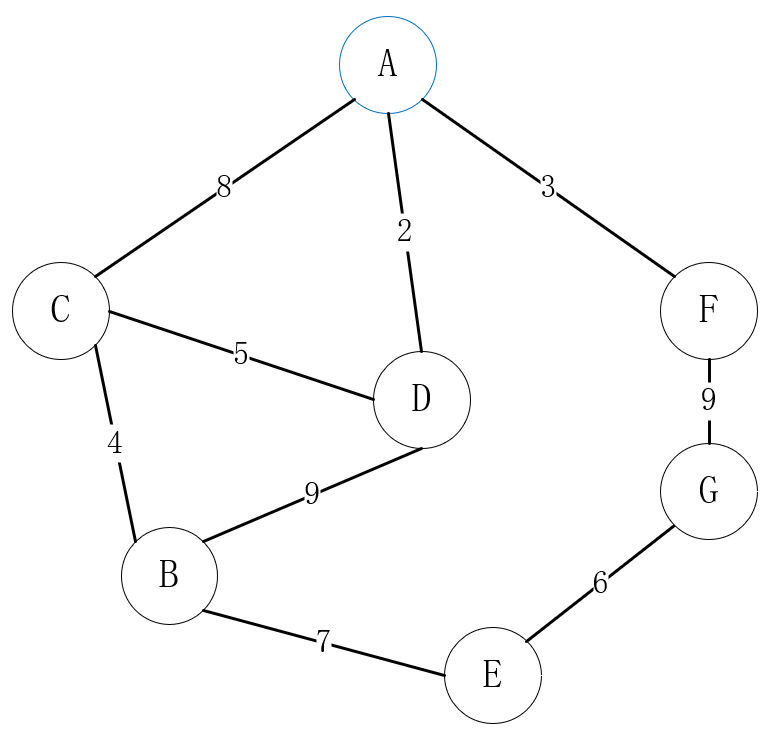上图就是生活中出行路线的选择映射到图形结构的案例。顶点作为站点，站点之间能够到达则拥有边，站点的之间的通行时间则是边的权值。   对于出行路线的选择，不同的人有不同的选择。其中有一种很常见的选择是要求出发地和目的地之间的总通行时间最短，而不在乎中途到底有几站。毕竟通行时间对于很多人来说是宝贵的！   这样的问题转转换为数学模型，就是求带权图的最短路径，就是求带权图形两顶点之间的权值最小的路径。即如果从图中某一顶点（源点）到达另一顶点（终点）的路径可能不止一条，如何找到一条路径使得沿此路径上各边的权值总和（称为路径长度）达到最小。   实际上最短路径有两重含义，一个两顶点之间的路径数量最少，另一个是两顶点之间的路径距离最短，本次主要解决路径距离最短的问题，即最小权值和。常见的解决算法一般是两种，迪杰斯特拉（Dijkstra）算法和弗洛伊德（Floyd）算法。
2 杰斯特拉（Dijkstra）算法
2.1 原理
迪杰斯特拉（Dijkstra）算法是由荷兰计算机科学家狄克斯特拉于1959 年提出的，因此又叫狄克斯特拉算法。是从一个顶点到其余各顶点的最短路径算法，解决的是寻找给定的加权图中指定顶点间最短路径问题的算法   Dijkstra算法并不是一下子就求出了起点到终点的最短路径，而是采用的是贪心算法策略，一步步求出它们之间顶点的最短路径，过程中都是基于已经求出的最短路径的基础上，求得更远顶点的最短路径，最终得到起点和终点的最短路径。   通用步骤如下：
指定两个集合S和U。S的作用是记录已求出最短路径的顶点，而U则是记录还未求出最短路径的顶点，以及这些顶点到起始顶点的权。指定一个起始顶点A，存入集合S中，其他顶点以及到顶点A的权存入集合U中，从U中找出并移除路径最短的顶点B，并将其加入到S中，并且更新U中对应的路径权值（更新源点将新加入节点作为中间节点到达其它节点的距离）；重复该操作直到遍历所有顶点，此时S中的集合就是起点A到其他各个顶点的最短路径。
迪杰斯特拉算法只支持非负权图，它计算的是单源最短路径，即单个源点到剩余节点的最短路径，时间复杂度为O(n²)，对稀疏图运行更快。如果想知道所有顶点到所有顶点的最短路径，那么等于在原有算法的基础上，再来一次循环，此时整个算法的时间复杂度就成了O(n3)。
2.2 案例分析该案例对应着下面实现代码中的案例，设起始点为A，初始化A到其他点的路径:{0, 99, 8, 2, 99, 3, 99}，初始化标志位数组{true, false, false, false, false, false, false}。   开始第一轮循环，排除已找到的路径，即排除0，寻找到A的最短路径，找到了A-D，即索引为3的顶点,设置对应位置的最短路径标志位为true，更新未获取最短路径的顶点的最短路径，因为其他已找到的顶点的最短路径已经找到了，这里只需要更新新找到的D可达的顶点路径到A点的最短路径，如果经过D点的路径比数组中已存在的最短路径小，那么更新值。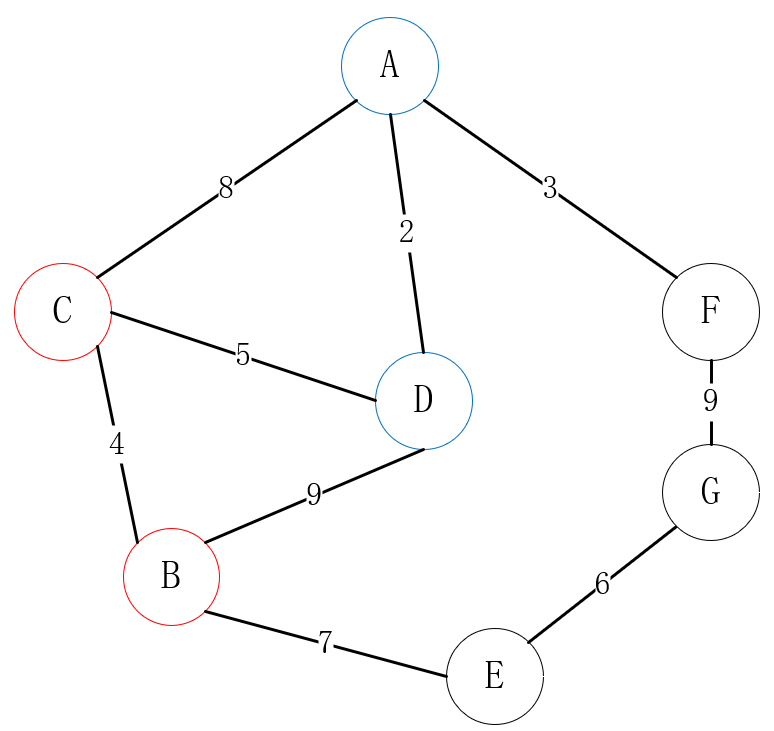这里D点可达C、B，D-C+A-D=7<8，因此更新A-C的最短路径为7；D-B+A-D=11<99，因此更新A-B的最短路径为11，第一轮循环结束，此时最短路径数组为{0, 11, 7, 2, 99, 3, 99}，标志位数组为{true, false, false, true, false, false, false}:   开始第二轮循环，排除已找到的路径，即排除0、2，寻找到A的最短路径，这里找到3，即索引为5的顶点，即A-F，设置对应位置的最短路径标志位为true，更新未获取最短路径的顶点的最短路径，因为其他已找到的顶点的最短路径已经找到了，这里只需要更新新找到的F可达的顶点路径到A点的最短路径，如果经过F点的路径比数组中已存在的最短路径小，那么更新值。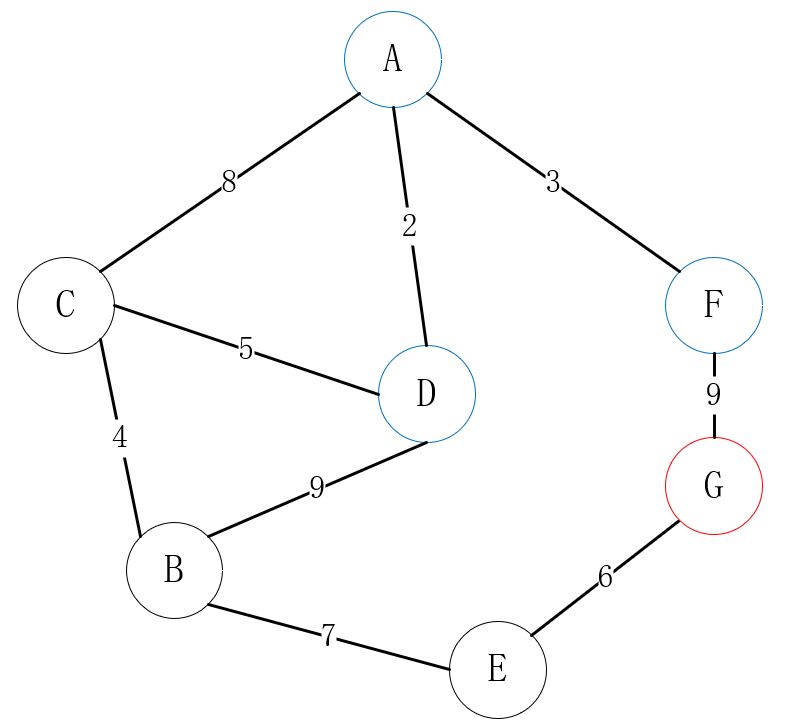这里F点可达G，F-G+A-F=12<99，因此更新A-G的最短路径为12，第二轮循环结束，此时最短路径数组为{0, 11, 7, 2, 99, 3, 12}，标志位数组为{true, false, false, true, false, true, false}。   开始第三轮循环，排除已找到的路径，即排除0、2、3，寻找到A的最短路径，这里找到7，即索引为2的顶点，即A-C，设置对应位置的最短路径标志位为true，更新未获取最短路径的顶点的最短路径，因为其他已找到的顶点的最短路径已经找到了，这里只需要更新新找到的C可达的顶点路径到A点的最短路径，如果经过C点的路径比数组中已存在的最短路径小，那么更新值。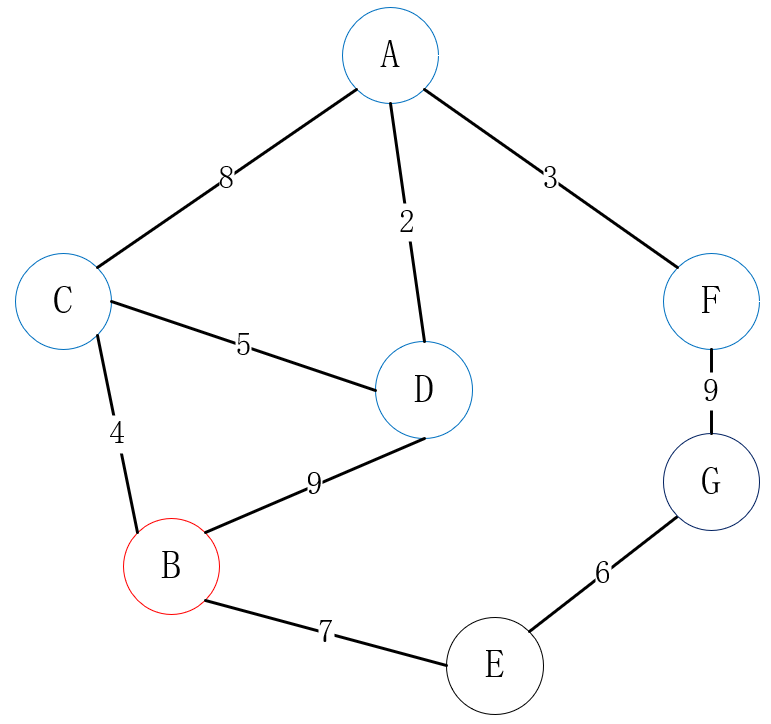这里C点可达B，C-B+A-C=11 = 11，因此不更新A-B的最短路径，第三轮循环结束，此时最短路径数组为{0, 11, 7, 2, 99, 3, 12}，标志位数组为{true, false, true, true, false, true, false}。   开始第四轮循环，排除已找到的路径，即排除0、2、3、7，寻找到A的最短路径，这里找到11，即索引为1的顶点，即A-B，设置对应位置的最短路径标志位为true，更新未获取最短路径的顶点的最短路径，因为其他已找到的顶点的最短路径已经找到了，这里只需要更新新找到的B可达的顶点路径到A点的最短路径，如果经过B点的路径比数组中已存在的最短路径小，那么更新值。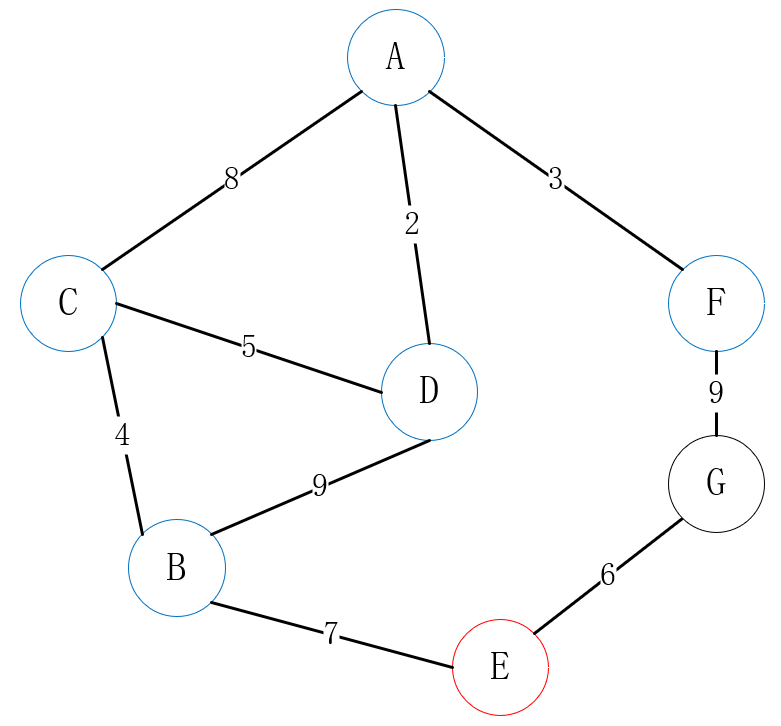这里B点可达E，B-E+A-B=18 < 99，因此更新A-E的最短路径为18，第四轮循环结束，此时最短路径数组为{0, 11, 7, 2, 18, 3, 12}，标志位数组为{true, true, true, true, false, true, false}。   开始第五轮循环，排除已找到的路径，即排除0、2、3、7、11，寻找到A的最短路径，这里找到12，即索引为6的顶点，即A-G，设置对应位置的最短路径标志位为true，更新未获取最短路径的顶点的最短路径，因为其他已找到的顶点的最短路径已经找到了，这里只需要更新新找到的G可达的顶点路径到A点的最短路径，如果经过G点的路径比数组中已存在的最短路径小，那么更新值。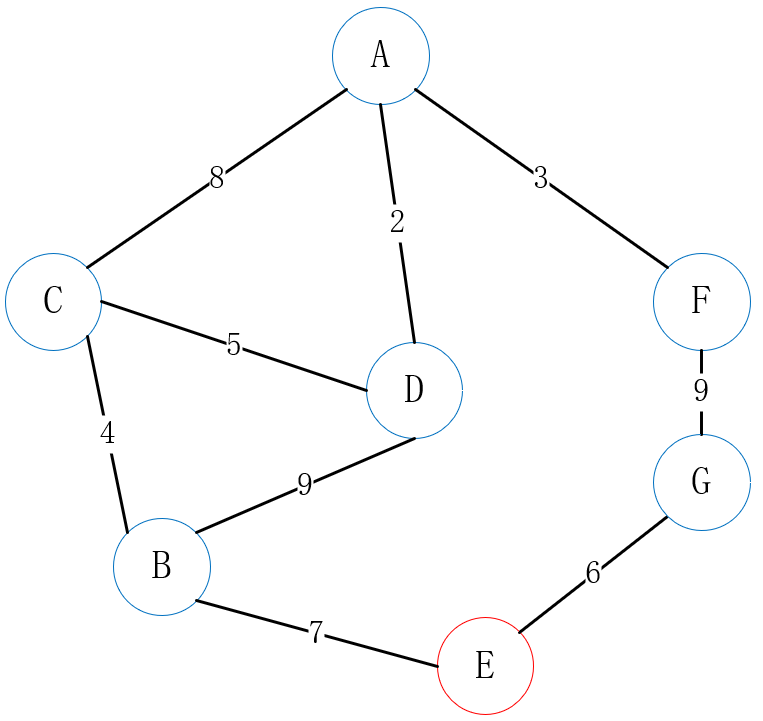排除已找到的顶点，这里G点可达E，G-E+A-G=18 = 18，因此不更新最短路径，第五轮循环结束，此时最短路径数组为{0, 11, 7, 2, 18, 3, 12}，标志位数组为{true, true, true, true, false, true, true}。   开始第六轮循环，排除已找到的路径，即排除0、2、3、7、11、12，寻找到A的最短路径，这里找到18，即索引为4的顶点，即A-E，设置对应位置的最短路径标志位为true，更新未获取最短路径的顶点的最短路径，因为其他已找到的顶点的最短路径已经找到了，这里只需要更新新找到的E可达的顶点路径到A点的最短路径，如果经过E点的路径比数组中已存在的最短路径小，那么更新值。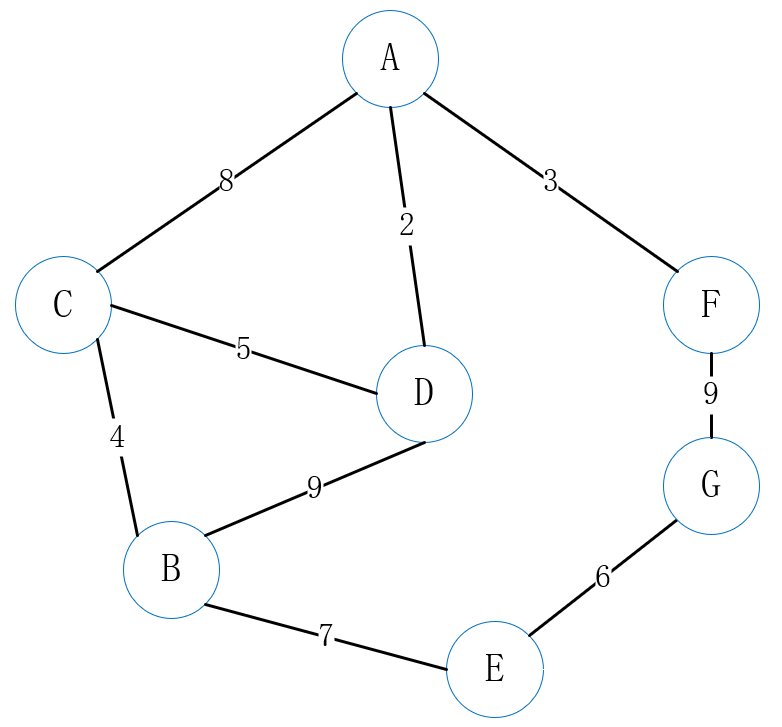排除已找到的顶点，这里不更新最短路径，第四轮循环结束，此时最短路径数组为{0, 11, 7, 2, 18, 3, 12}，标志位数组为{true, true, true, true, true, true, true}。   此时大循环结束，Dijkstra算法结束，顶点A到各个顶点的最短路径已经找到，即A到{A,B,C,D,E,F,G}的最短路径为{0, 11, 7, 2, 18, 3, 12}。
3 弗洛伊德（Floyd）算法
3.1 原理
弗洛伊德（Floyd）算法又称插点法，是一种利用动态规划的思想寻找给定的加权图中多源点之间最短路径的算法。算出来的结果是所有的节点到其余各节点之间的最短距离。   通用步骤如下：
设图顶点数为N。首先需要准备一个长度为N的距离矩阵S，矩阵S中的元素a[i][j]=sum的表示顶点i到顶点j的最短路径为sum；然后对S矩阵进行初始化，距离矩阵S中顶点a[i][j]的值为顶点i到顶点j的直接权值；然后对S矩阵循环进行N次更新，在第k次更新时，如果S矩阵的a[i][j] > a[i][k]+a[k][j]，那么更新a[i][j]=a[i][k]+a[k][j]。循环更新完毕，则算法完成，所有的节点到其余各节点之间的最短距离已经找到了。
相比于Dijkstra 算法，Floyd算法支持带有负权边的图，但是不能解决带有“负权回路”（或者叫“负权环”）的图，实际上如果一个图中带有“负权回路”那么这个图则没有最短路径。   Floyd算法的时间复杂度同样是时间复杂度O(n3)，空间复杂度是O(n2)，代码非常简单，但是思想相却是非常的巧妙，将所有的可能都枚举出来一一对比，取最小值，这样最终会得到最小值。
3.2 案例分析
该案例对应着下面实现代码中的案例：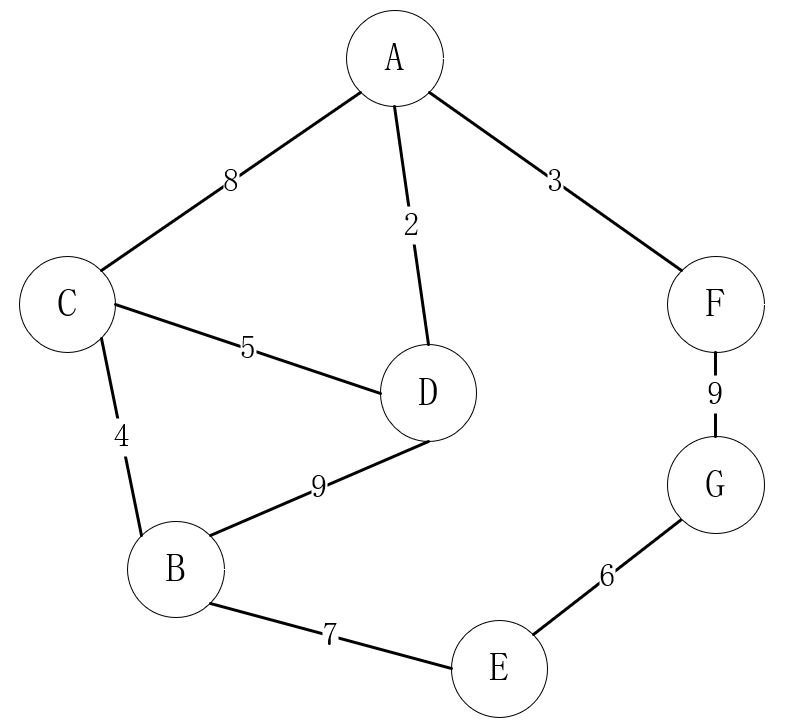首先初始化距离矩阵S如下：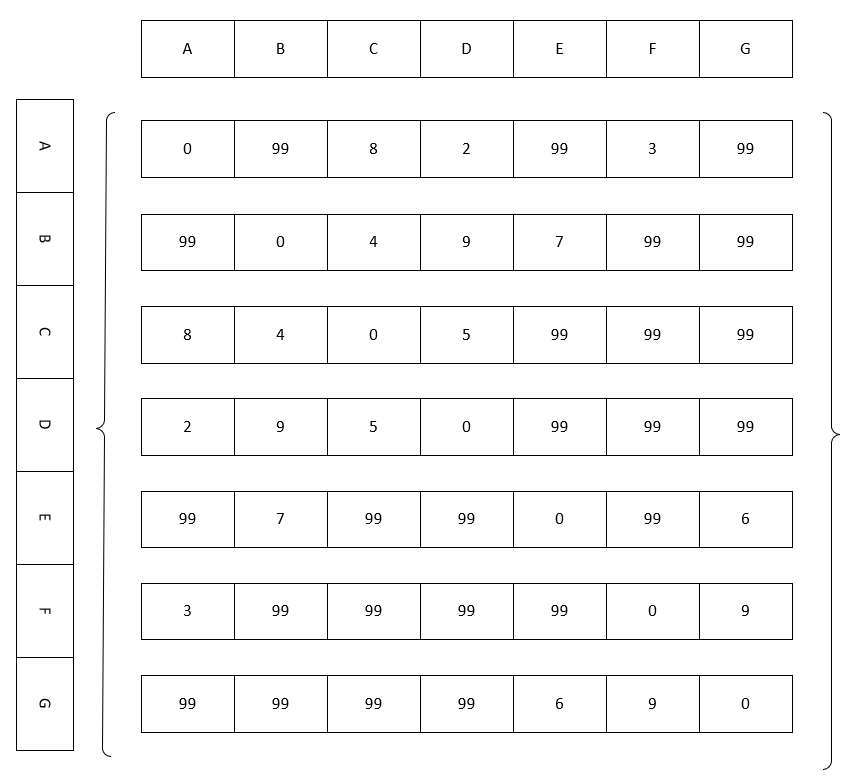然后就是三层嵌套循环，开始第一轮大循环，即当k=0，循环遍历S矩阵，判断是否小于 shortestPath[i][j]，即所有的路径都经过A点中转，如果经过A中转后的路径shortestPath[i] + shortestPath[k]< shortestPath[i][j]，自然更新路径：shortestPath[i][j]= shortestPath[i] + shortestPath[k]。一轮大循环之后的数组如下：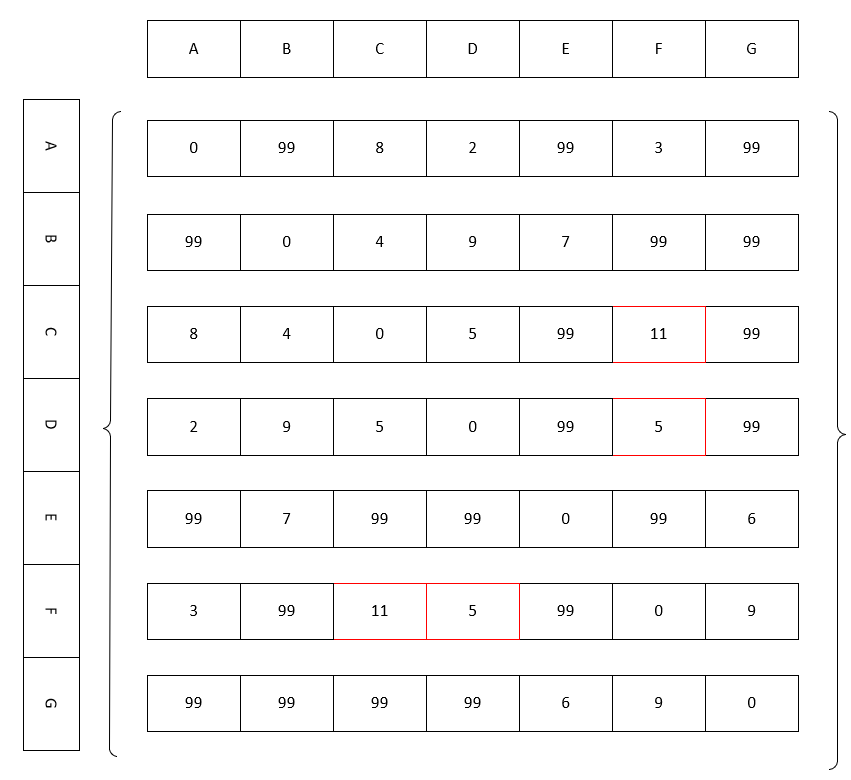然后经过一共经过N次的大循环，表示经过所有的顶点，最终取得的矩阵如下：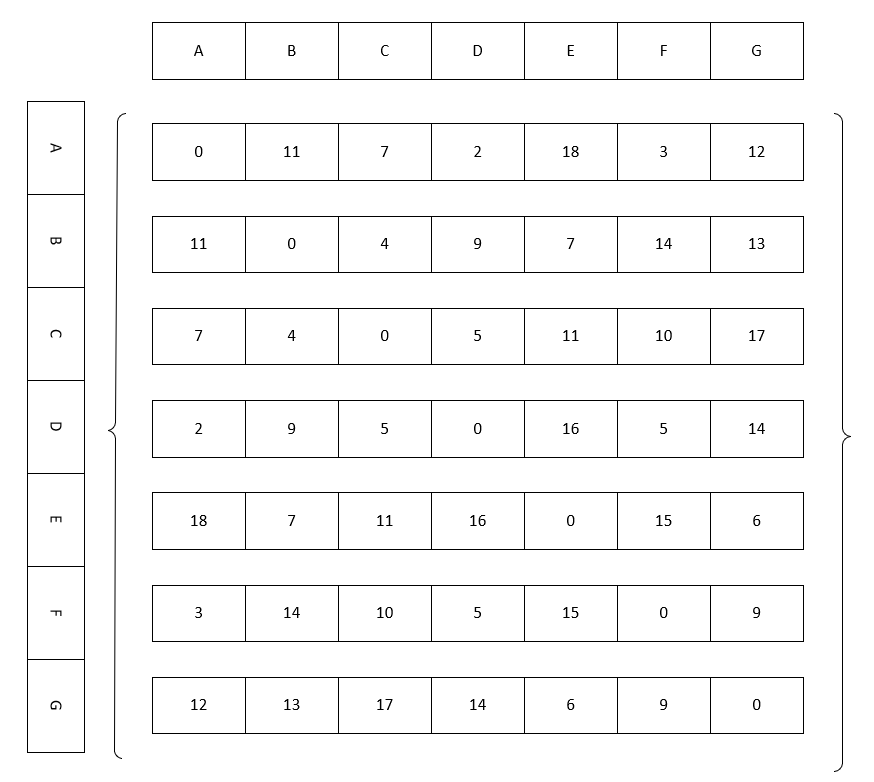4 邻接矩阵加权图实现
这里的实现能够构造一个基于邻接矩阵实现无向加权图的类，并且提供深度优先遍历和广度优先遍历的方法，提供获取边集数组的方法，提供Prim和Kruskal两种求最小生成树的方法，提供Dijkstra和Floyd两种求最短路径的方法。
/**
* 无向加权图邻接矩阵实现
*
* @author lx
*/
public class MatrixDijkstraAndFloyd<E> {

/**
* 顶点数组
*/
private Object[] vertexs;

/**
* 邻接矩阵
*/
private int[][] matrix;

/**
*
*/
private Edge<E>[] edges;

/**
* 由于是加权图,这里设置一个边的权值上限,任何边的最大权值不能大于等于该值，在实际应用中，该值应该根据实际情况确定
*/
private static final int NO_EDGE = 99;

/**
* 边对象,具有权值,在构建加权无向图时使用
*/
private static class Edge<E> {

private E from;
private E to;
private int weight;

public Edge(E from, E to, int weight) {
this.from = from;
this.to = to;
this.weight = weight;
}

@Override
public String toString() {
return "Edge{" +
"from=" + from +
", to=" + to +
", weight=" + weight +
'}';
}
}

/**
* 创建无向加权图
*
* @param vertexs 顶点数组
* @param edges   边对象数组
*/
public MatrixDijkstraAndFloyd(Object[] vertexs, Edge<E>[] edges) {
//初始化边数组
this.edges = edges;
// 初始化顶点数组,并添加顶点
this.vertexs = Arrays.copyOf(vertexs, vertexs.length);
// 初始化边矩阵,并预先填充边信息
this.matrix = new int[vertexs.length][vertexs.length];
for (int i = 0; i < vertexs.length; i++) {
for (int j = 0; j < vertexs.length; j++) {
if (i == j) {
this.matrix[i][j] = 0;
} else {
this.matrix[i][j] = NO_EDGE;
}
}
}
for (Edge<E> edge : edges) {
// 读取一条边的起始顶点和结束顶点索引值
int p1 = getPosition(edge.from);
int p2 = getPosition(edge.to);
//对称的两个点位都置为edge.weight,无向图可以看作相互可达的有向图
this.matrix[p1][p2] = edge.weight;
this.matrix[p2][p1] = edge.weight;
}
}

/**
* 获取某条边的某个顶点所在顶点数组的索引位置
*
* @param e 顶点的值
* @return 所在顶点数组的索引位置, 或者-1 - 表示不存在
*/
private int getPosition(E e) {
for (int i = 0; i < vertexs.length; i++) {
if (vertexs[i] == e) {
return i;
}
}
return -1;
}

/**
* 深度优先搜索遍历图,类似于树的前序遍历,
*/
public void DFS() {
//新建顶点访问标记数组,对应每个索引对应相同索引的顶点数组中的顶点
boolean[] visited = new boolean[vertexs.length];
//初始化所有顶点都没有被访问
for (int i = 0; i < vertexs.length; i++) {
visited[i] = false;
}
System.out.println("DFS: ");
System.out.print("\t");
for (int i = 0; i < vertexs.length; i++) {
if (!visited[i]) {
DFS(i, visited);
}
}
System.out.println();
}

/**
* 深度优先搜索遍历图的递归实现,类似于树的先序遍历
* 因此模仿树的先序遍历,同样借用栈结构,这里使用的是方法的递归,隐式的借用栈
*
* @param i       顶点索引
* @param visited 访问标志数组
*/
private void DFS(int i, boolean[] visited) {
visited[i] = true;
System.out.print(vertexs[i] + " ");
// 遍历该顶点的所有邻接点。若该邻接点是没有访问过，那么继续递归遍历领接点
for (int w = firstVertex(i); w >= 0; w = nextVertex(i, w)) {
if (!visited[w]) {
DFS(w, visited);
}
}
}

/**
* 广度优先搜索图,类似于树的层序遍历
* 因此模仿树的层序遍历,同样借用队列结构
*/
public void BFS() {
// 辅组队列
//新建顶点访问标记数组,对应每个索引对应相同索引的顶点数组中的顶点
boolean[] visited = new boolean[vertexs.length];
for (int i = 0; i < vertexs.length; i++) {
visited[i] = false;
}
System.out.println("BFS: ");
System.out.print("\t");
for (int i = 0; i < vertexs.length; i++) {
if (!visited[i]) {
visited[i] = true;
System.out.print(vertexs[i] + " ");
}
//j索引出队列
//继续访问j的邻接点
for (int k = firstVertex(j); k >= 0; k = nextVertex(j, k)) {
if (!visited[k]) {
visited[k] = true;
System.out.print(vertexs[k] + " ");
//继续入队列
}
}
}
}
System.out.println();
}

/**
* 返回顶点v的第一个邻接顶点的索引，失败则返回-1
*
* @param v 顶点v在数组中的索引
* @return 返回顶点v的第一个邻接顶点的索引，失败则返回-1
*/
private int firstVertex(int v) {
//如果索引超出范围,则返回-1
if (v < 0 || v > (vertexs.length - 1)) {
return -1;
}
/*根据邻接矩阵的规律:顶点索引v对应着边二维矩阵的matrix[v][i]一行记录
* 从i=0开始*/
for (int i = 0; i < vertexs.length; i++) {
if (matrix[v][i] != 0 && matrix[v][i] != NO_EDGE) {
return i;
}
}
return -1;
}

/**
* 返回顶点v相对于w的下一个邻接顶点的索引，失败则返回-1
*
* @param v 顶点索引
* @param w 第一个邻接点索引
* @return 返回顶点v相对于w的下一个邻接顶点的索引，失败则返回-1
*/
private int nextVertex(int v, int w) {
//如果索引超出范围,则返回-1
if (v < 0 || v > (vertexs.length - 1) || w < 0 || w > (vertexs.length - 1)) {
return -1;
}
/*根据邻接矩阵的规律:顶点索引v对应着边二维矩阵的matrix[v][i]一行记录
* 由于邻接点w的索引已经获取了,所以从i=w+1开始寻找*/
for (int i = w + 1; i < vertexs.length; i++) {
if (matrix[v][i] != 0 && matrix[v][i] != NO_EDGE) {
return i;
}
}
return -1;
}

/**
* 输出图
*
* @return 输出图字符串
*/
@Override
public String toString() {
StringBuilder stringBuilder = new StringBuilder();
for (int i = 0; i < vertexs.length; i++) {
for (int j = 0; j < vertexs.length; j++) {
stringBuilder.append(matrix[i][j]).append("\t");
}
stringBuilder.append("\n");
}
return stringBuilder.toString();
}

/**
* Prim算法求最小生成树
*/
public void prim() {
System.out.println("prim: ");
//对应节点应该被连接的前驱节点,用来输出
//默认为0,即前驱结点为第一个节点
int[] mid = new int[matrix.length];
//如果某顶点作为末端顶点被连接，对应位置应该为true
//第一个顶点默认被连接
boolean[] connected = new boolean[matrix.length];
connected = true;
//存储未连接顶点到已连接顶点的最短距离(最小权)
int[] dis = new int[matrix.length];
//首先将矩阵第一行即其他顶点到0索引顶点的权值拷贝进去
System.arraycopy(matrix, 0, dis, 0, matrix.length);
//存储路径长度
int sum = 0;
//最小权值
int min;
/*默认第一个顶点已经找到了,因此最多还要需要大循环n-1次*/
for (int k = 1; k < matrix.length; k++) {
min = NO_EDGE;
//最小权值的顶点的索引
int minIndex = 0;
/*寻找权值最小的且未被连接的顶点索引*/
for (int i = 1; i < matrix.length; i++) {
//排除已连接的顶点,排除权值等于0的值,这里权值等于0表示已生成的最小生成树的顶点都未能与该顶点连接
if (!connected[i] && dis[i] != 0 && dis[i] < min) {
min = dis[i];
minIndex = i;
}
}
//如果没找到,那么该图可能不是连通图,直接返回了,此时最小生成树没啥意义
if (minIndex == 0) {
return;
}
//权值和增加
sum += min;
//该新连接顶点对应的索引值变成true,表示已被连接,后续判断时跳过该顶点
connected[minIndex] = true;
//输出对应的前驱顶点到该最小顶点的权值
System.out.println("\t" + vertexs[mid[minIndex]] + " ---> " + vertexs[minIndex] + " 权值:" + min);
/*在新顶点minIndex加入之前的其他所有顶点到连接顶点最小的权值已经计算过了
因此只需要更新其他未连接顶点到新连接顶点minIndex是否还有更短的权值,有的话就更新找到距离已连接的顶点权最小的顶点*/
for (int i = 1; i < matrix.length; i++) {
//如果该顶点未连接
if (!connected[i]) {
/*如果新顶点到未连接顶点i的权值不为0,并且比原始顶点到未连接顶点i的权值还要小,那么更新对应位置的最小权值*/
if (matrix[minIndex][i] != 0 && dis[i] > matrix[minIndex][i]) {
//更新最小权值
dis[i] = matrix[minIndex][i];
//更新前驱节点索引为新加入节点索引
mid[i] = minIndex;
}
}

}
}
System.out.println("\t" + "sum: " + sum);
}

/**
* Kruskal算法求最小生成树传统实现,要求知道边集数组,和顶点数组
*/
public void kruskal() {
System.out.println("Kruskal: ");
//由于创建图的时候保存了边集数组,这里直接使用就行了
//Edge[] edges = getEdges();
//this.edges=edges;
//对边集数组进行排序
Arrays.sort(this.edges, Comparator.comparingInt(o -> o.weight));
// 用于保存已有最小生成树中每个顶点在该最小树中的最终终点的索引
int[] vends = new int[this.edges.length];
//能够知道终点索引范围是[0,this.edges.length-1],因此填充edges.length表示没有终点
Arrays.fill(vends, this.edges.length);
int sum = 0;
for (Edge<E> edge : this.edges) {
// 获取第i条边的起点索引from
int from = getPosition(edge.from);
// 获取第i条边的终点索引to
int to = getPosition(edge.to);
// 获取顶点from在"已有的最小生成树"中的终点
int m = getEndIndex(vends, from);
// 获取顶点to在"已有的最小生成树"中的终点
int n = getEndIndex(vends, to);
// 如果m!=n，意味着没有形成环路,则可以添加,否则直接跳过,进行下一条边的判断
if (m != n) {
//添加设置原始终点索引m在已有的最小生成树中的终点为n
vends[m] = n;
System.out.println("\t" + vertexs[from] + " ---> " + vertexs[to] + " 权值:" + edge.weight);
sum += edge.weight;
}
}
System.out.println("\t" + "sum: " + sum);
//System.out.println(Arrays.toString(this.edges));
}

/**
* 获取顶点索引i的终点如果没有终点则返回顶点索引本身
*
* @param vends 顶点在最小生成树中的终点
* @param i     顶点索引
* @return 顶点索引i的终点如果没有终点则返回顶点索引本身
*/
private int getEndIndex(int[] vends, int i) {
//这里使用循环查找的逻辑,寻找的是最终的终点
while (vends[i] != this.edges.length) {
i = vends[i];
}
return i;
}

/**
* 如果没有现成的边集数组，那么根据邻接矩阵结构获取图中的边集数组
*
* @return 图的边集数组
*/
private Edge[] getEdges() {
List<Edge> edges = new ArrayList<>();
/*遍历矩阵数组 只需要遍历一半就行了*/
for (int i = 0; i < vertexs.length; i++) {
for (int j = i + 1; j < vertexs.length; j++) {
//如果存在边
if (matrix[i][j] != NO_EDGE && matrix[i][j] != 0) {
//edges[index++] = new Edge(vertexs[i], vertexs[j], matrix[i][j]);
}
}
}
return edges.toArray(new Edge);
}

/**
* Kruskal结合Prim算法.不需要知道边集,只需要矩阵数组,和顶点数组
* 同样是求最小权值的边,但是有一个默认起点顶点,该起点可以是要求[0,顶点数量-1]之间的任意值，同时查找最小权的边。
* 可能会有Bug,目前未发现
*/
public void kruskalAndPrim() {
System.out.println("kruskalAndPrim: ");
//已经找到的边携带的顶点对应的索引将变为true,其余未找到边对应的顶点将是false
boolean[] connected = new boolean[matrix.length];
//这里选择第一个顶点为起点,表示以该顶点开始寻找包含该顶点的最小边
connected = true;
int sum = 0, n1 = 0, n2 = 0;
//最小权值
int min;
while (true) {
min = NO_EDGE;
/*找出所有带有已找到顶点的边中,最小权值的边,只需要寻找对称矩阵的一半即可*/
//第一维
for (int i = 0; i < matrix.length; i++) {
//第二维
for (int j = i + 1; j < matrix.length; j++) {
//排除等于0的,排除两个顶点都找到了的,这里实际上已经隐含了排除环的逻辑,如果某条边的两个顶点都找到了,那么如果算上该条边,肯定会形成环
//寻找剩下的最小的权值的边
if (matrix[i][j] != 0 && connected[i] != connected[j] && matrix[i][j] < min) {
min = matrix[i][j];
n1 = i;
n2 = j;
}
}
}
//如果没找到最小权值,该图可能不是连通图,或者已经寻找完毕,直接返回
if (min == NO_EDGE) {
System.out.println("\t" + "sum:" + sum);
return;
}
//已经找到的边对应的两个顶点都置为true
connected[n1] = true;
connected[n2] = true;
//输出找到的边和最小权值
System.out.println("\t" + vertexs[n1] + " ---> " + vertexs[n2] + " 权值:" + min);
sum += min;
}
}

/**
* Dijkstra算法求最短路径。
*
* @param start 起始顶点索引。即计算"顶点vs"到其它顶点的最短路径。
*/
public void dijkstra(int start) {
checkIndex(start);
int[] shortestPathance = getShortestDistance(start, vertexs.length);
// 打印Dijkstra最短路径的结果
System.out.println("Dijkstra(" + vertexs[start] + "):");
for (int i = 0; i < vertexs.length; i++) {
System.out.println("\t(" + vertexs[start] + " ---> " + vertexs[i] + ")最短路径:" + shortestPathance[i]);
}
}

/**
* Dijkstra算法求最短路径
*
* @param start 起始点
* @param end   终点,如果end=vertexs.length说明是遍历查找所有的最短路径
* @return 起始顶点到其他点或者指定点的最短权值
*/
private int[] getShortestDistance(int start, int end) {
/*1、该数组存放起始顶点到其他点的权值*/
int[] shortestPathance = new int[vertexs.length];
//初始化数据
//首先设置起始点到顶点i到的最短路径为起始点到顶点i的权。
System.arraycopy(matrix[start], 0, shortestPathance, 0, matrix.length);

/*2、标志位数组.某个位置如果为true表示对应位置的顶点到起始顶点的最短路径已成功获取。*/
boolean[] shortest = new boolean[vertexs.length];
//首先设置起始点到自己的的路径已经找到了,为0
shortest[start] = true;

/*3、最多遍历vertexs.length-1次；每次找出起始点到一个顶点的最短路径。*/
int k;
int min;
for (int i = 1; i < vertexs.length; i++) {
k = 0;
// 寻找当前最小的路径；
min = NO_EDGE;
for (int j = 0; j < vertexs.length; j++) {
//排除已经找到的最短路径之后，找到离start最近的顶点(k)。
if (!shortest[j] && shortestPathance[j] < min) {
min = shortestPathance[j];
k = j;
}
}
//先设置起始点到新顶点k的最短路径已经找到
shortest[k] = true;
if (end != vertexs.length && k == end) {
break;
}
//更新未获取最短路径的顶点的最短路径,因为其他已找到的顶点的最短路径已经找到了,这里指需要更新新加入的已找到的可达顶点的路径.
for (int j = 0; j < vertexs.length; j++) {
int tmp = matrix[k][j];
//排除已经找到的最短路径，排除未连接的路径，排除等于0的路径（连接自己）之后
//找到离start最如果新的最短路径比以前的最短路径还要短，则更新最短路径。
if (!shortest[j] && tmp != NO_EDGE && tmp != 0 && ((tmp = min + tmp) < shortestPathance[j])) {
shortestPathance[j] = tmp;
}
}
}
return shortestPathance;
}

/**
* 索引检查
*
* @param index 多个索引
*/
private void checkIndex(int... index) {
for (int i : index) {
if (i < 0 || i >= vertexs.length) {
throw new ArrayIndexOutOfBoundsException("索引越界:" + i);
}
}
}

/**
* Dijkstra算法求最短路径。
*
* @param start 起始顶点索引。
* @param end   结束点索引
*/
public void dijkstra(int start, int end) {
checkIndex(start, end);
int[] shortestPathance = getShortestDistance(start, end);
// 打印Dijkstra最短路径的结果
System.out.println("Dijkstra(" + vertexs[start] + " ---> " + vertexs[end] + ")最短路径:" + shortestPathance[end]);
}

/**
* Floyd算法获取所有顶点到所有顶点的最短路径,代码很简单,思想很巧妙
*/
public void floyd() {
//路径矩阵(两顶点最短路径,即最小权值)
int[][] shortestPath = new int[matrix.length][matrix.length];
/*初始化数据*/
for (int i = 0; i < matrix.length; i++) {
System.arraycopy(matrix[i], 0, shortestPath[i], 0, vertexs.length);
}
// 计算最短路径
for (int k = 0; k < matrix.length; k++) {
for (int i = 0; i < matrix.length; i++) {
for (int j = 0; j < matrix.length; j++) {
//要求经过下标k顶点的两个路径都不能等于NO_EDGE,否则就是没有路径,NO_EDGE应该选取的足够的大,否则可能出错
int tmp = (shortestPath[i][k] == NO_EDGE || shortestPath[k][j] == NO_EDGE) ? NO_EDGE : (shortestPath[i][k] + shortestPath[k][j]);
// 如果经过下标为k顶点路径比原两点间路径更短，则更新shortestPath[i][j]
if (shortestPath[i][j] > tmp) {
// i到j最短路径对应的值设为经过k的更小的一个
shortestPath[i][j] = tmp;
}

}
}
}
/*输出路径矩阵*/
System.out.println("Floyd: ");
for (int i = 0; i < matrix.length; i++) {
for (int j = 0; j < matrix.length; j++) {
System.out.print("\t" + shortestPath[i][j]);
}
System.out.println();
}
}

public static void main(String[] args) {
//顶点数组
Character[] vexs = {'A', 'B', 'C', 'D', 'E', 'F', 'G'};
//边数组,加权值
Edge[] edges = {
new Edge<>('A', 'C', 8),
new Edge<>('D', 'A', 2),
new Edge<>('A', 'F', 3),
new Edge<>('B', 'C', 4),
new Edge<>('C', 'D', 5),
new Edge<>('E', 'G', 6),
new Edge<>('E', 'B', 7),
new Edge<>('D', 'B', 9),
new Edge<>('F', 'G', 9)};

//构建图
MatrixDijkstraAndFloyd<Character> matrixDijkstraAndFloyd = new MatrixDijkstraAndFloyd<Character>(vexs, edges);
//输出图
System.out.println(matrixDijkstraAndFloyd);
//深度优先遍历
matrixDijkstraAndFloyd.DFS();
//广度优先遍历
matrixDijkstraAndFloyd.BFS();
//Prim算法输出最小生成树
matrixDijkstraAndFloyd.prim();
//Kruskal算法输出最小生成树
matrixDijkstraAndFloyd.kruskal();
//Kruskal算法结合Prim算法输出最小生成树,可能会有Bug,目前未发现
matrixDijkstraAndFloyd.kruskalAndPrim();

// Dijkstra算法获取某个索引的顶点到其它各个顶点的最短距离
// 这里参数是索引,也可以是一个顶点,需要稍微修改代码获取顶点的索引,比较简单这里就不做了
matrixDijkstraAndFloyd.dijkstra(0);
// Dijkstra算法获取一个顶点到另一个顶点的最短距离
matrixDijkstraAndFloyd.dijkstra(2, 0);

// Floyd算法获取所有顶点到所有顶点的最短路径
matrixDijkstraAndFloyd.floyd();
}
}

5 邻接表加权图实现
这里的实现能够构造一个基于邻接表实现无向加权图的类；并且提供深度优先遍历和广度优先遍历的方法，提供获取边集数组的方法，提供Prim和Kruskal两种求最小生成树的方法，，提供Dijkstra和Floyd两种求最短路径的方法。
/**
* 无向加权图邻接表实现
*
* @author lx
*/
public class ListDijkstraAndFloyd<E> {
/**
* 顶点类
*
* @param <E>
*/
private class Node<E> {
/**
* 顶点信息
*/
E data;
/**
* 指向第一条依附该顶点的边
*/
LNode firstLNode;

public Node(E data, LNode firstLNode) {
this.data = data;
this.firstLNode = firstLNode;
}
}

/**
* 边表节点类
*/
private class LNode {
/**
* 该边所指向的顶点的索引位置
*/
int vertex;
/**
* 该边的权值
*/
int weight;
/**
* 指向下一条边的指针
*/
LNode nextLNode;
}

/**
* 边对象,具有权值,在构建加权无向图时使用
*/
private static class Edge<E> {

private E from;
private E to;
private int weight;

public Edge(E from, E to, int weight) {
this.from = from;
this.to = to;
this.weight = weight;
}

@Override
public String toString() {
return "Edge{" +
"from=" + from +
", to=" + to +
", weight=" + weight +
'}';
}
}

/**
* 顶点数组
*/
private Node<E>[] vertexs;

/**
* 边数组
*/
private Edge<E>[] edges;

/**
* 由于是加权图,这里设置一个边的权值上限,任何边的最大权值不能大于等于该值，在实际应用中，该值应该根据实际情况确定
*/
private static final int NO_EDGE = 99;

/**
* 创建无向加权图
*
* @param vexs  顶点数组
* @param edges 边二维数组
*/
public ListDijkstraAndFloyd(E[] vexs, Edge<E>[] edges) {
this.edges = edges;
/*初始化顶点数组,并添加顶点*/
vertexs = new Node[vexs.length];
for (int i = 0; i < vertexs.length; i++) {
vertexs[i] = new Node<>(vexs[i], null);
}
/*初始化边表,并添加边节点到边表尾部,即采用尾插法*/
for (Edge<E> edge : edges) {
// 读取一条边的起始顶点和结束顶点索引值
int p1 = getPosition(edge.from);
int p2 = getPosition(edge.to);
int weight = edge.weight;
/*这里需要相互添加边节点,无向图可以看作相互可达的有向图*/
// 初始化lnode1边节点
LNode lnode1 = new LNode();
lnode1.vertex = p2;
lnode1.weight = weight;
// 将LNode链接到"p1所在链表的末尾"
if (vertexs[p1].firstLNode == null) {
vertexs[p1].firstLNode = lnode1;
} else {
}
// 初始化lnode2边节点
LNode lnode2 = new LNode();
lnode2.vertex = p1;
lnode2.weight = weight;
// 将lnode2链接到"p2所在链表的末尾"
if (vertexs[p2].firstLNode == null) {
vertexs[p2].firstLNode = lnode2;
} else {
}
}
}

/**
* 获取某条边的某个顶点所在顶点数组的索引位置
*
* @param e 顶点的值
* @return 所在顶点数组的索引位置, 或者-1 - 表示不存在
*/
private int getPosition(E e) {
for (int i = 0; i < vertexs.length; i++) {
if (vertexs[i].data == e) {
return i;
}
}
return -1;
}

/**
* 将lnode节点链接到边表的最后,采用尾插法
*
* @param first 边表头结点
* @param node  将要添加的节点
*/
private void linkLast(LNode first, LNode node) {
while (true) {
if (first.vertex == node.vertex) {
return;
}
if (first.nextLNode == null) {
break;
}
first = first.nextLNode;
}
first.nextLNode = node;
}

@Override
public String toString() {
StringBuilder stringBuilder = new StringBuilder();
for (int i = 0; i < vertexs.length; i++) {
stringBuilder.append(i).append("(").append(vertexs[i].data).append("): ");
LNode node = vertexs[i].firstLNode;
while (node != null) {
stringBuilder.append(node.vertex).append("(").append(vertexs[node.vertex].data).append("-").append(node.weight).append(")");
node = node.nextLNode;
if (node != null) {
stringBuilder.append("->");
} else {
break;
}
}
stringBuilder.append("\n");
}
return stringBuilder.toString();
}

/**
* 深度优先搜索遍历图的递归实现,类似于树的先序遍历
* 因此模仿树的先序遍历,同样借用栈结构,这里使用的是方法的递归,隐式的借用栈
*
* @param i       顶点索引
* @param visited 访问标志数组
*/
private void DFS(int i, boolean[] visited) {
//索引索引标记为true ,表示已经访问了
visited[i] = true;
System.out.print(vertexs[i].data + " ");
//获取该顶点的边表头结点
LNode node = vertexs[i].firstLNode;
//循环遍历该顶点的邻接点,采用同样的方式递归搜索
while (node != null) {
if (!visited[node.vertex]) {
DFS(node.vertex, visited);
}
node = node.nextLNode;
}
}

/**
* 深度优先搜索遍历图,类似于树的前序遍历,
*/
public void DFS() {
//新建顶点访问标记数组,对应每个索引对应相同索引的顶点数组中的顶点
boolean[] visited = new boolean[vertexs.length];
//初始化所有顶点都没有被访问
for (int i = 0; i < vertexs.length; i++) {
visited[i] = false;
}
System.out.println("DFS: ");
System.out.print("\t");
/*循环搜索*/
for (int i = 0; i < vertexs.length; i++) {
//如果对应索引的顶点的访问标记为false,则搜索该顶点
if (!visited[i]) {
DFS(i, visited);
}
}
/*走到这一步,说明顶点访问标记数组全部为true,说明全部都访问到了,深度搜索结束*/
System.out.println();
}

/**
* 广度优先搜索图,类似于树的层序遍历
* 因此模仿树的层序遍历,同样借用队列结构
*/
public void BFS() {
// 辅组队列
//新建顶点访问标记数组,对应每个索引对应相同索引的顶点数组中的顶点
boolean[] visited = new boolean[vertexs.length];
//初始化所有顶点都没有被访问
for (int i = 0; i < vertexs.length; i++) {
visited[i] = false;
}
System.out.println("BFS: ");
System.out.print("\t");
for (int i = 0; i < vertexs.length; i++) {
//如果访问方剂为false,则设置为true,表示已经访问,然后开始访问
if (!visited[i]) {
visited[i] = true;
System.out.print(vertexs[i].data + " ");
}
//判断队列是否有值,有就开始遍历
//出队列
LNode node = vertexs[j].firstLNode;
while (node != null) {
int k = node.vertex;
if (!visited[k]) {
visited[k] = true;
System.out.print(vertexs[k].data + " ");
//继续入队列
}
node = node.nextLNode;
}
}
}
System.out.println();
}

/**
* Prim算法求最小生成树
*/
public void prim() {
System.out.println("prim: ");
//对应节点应该被连接的前驱节点,用来输出
//默认为0,即前驱结点为第一个节点
int[] mid = new int[vertexs.length];
int start = 0;
int min, tmp, sum = 0;
int num = vertexs.length;

//顶点间边的权值
//存储未连接顶点到已连接顶点的最短距离(最小权)
int[] dis = new int[num];

// 初始化"顶点的权值数组"，
// 将每个顶点的权值初始化为"第start个顶点"到"该顶点"的权值。
//首先将其他顶点到0索引顶点的权值存储进去
for (int i = 0; i < num; i++) {
dis[i] = getWeight(start, i);
}
//如果某顶点作为末端顶点被连接，对应位置应该为true
//第一个顶点默认被连接
boolean[] connected = new boolean[vertexs.length];
connected = true;
/*默认第一个顶点已经找到了,因此最多还要需要大循环n-1次*/
for (int k = 1; k < num; k++) {
min = NO_EDGE;
//最小权值的顶点的索引
int minIndex = 0;
// 在未被加入到最小生成树的顶点中，找出权值最小的顶点。
for (int i = 1; i < vertexs.length; i++) {
//排除已连接的顶点,排除权值等于0的值,因为这里默认顶点指向自己的权值为0
if (!connected[i] && dis[i] != 0 && dis[i] < min) {
min = dis[i];
minIndex = i;
}
}
//如果没找到,那么该图可能不是连通图,直接返回了,此时最小生成树没啥意义
if (minIndex == 0) {
return;
}
//权值和增加
sum += min;
//该新连接顶点对应的索引值变成true,表示已被连接,后续判断时跳过该顶点
connected[minIndex] = true;
//输出对应的前驱顶点到该最小顶点的权值
System.out.println("\t" + vertexs[mid[minIndex]].data + " ---> " + vertexs[minIndex].data + " 权值:" + min);
/*在新顶点minIndex加入之前的其他所有顶点到连接顶点最小的权值已经计算过了
因此只需要更新其他顶点到新连接顶点minIndex是否还有更短的权值,有的话就更新找到距离已连接的顶点权最小的顶点*/
for (int i = 1; i < num; i++) {
//如果该顶点未连接
if (!connected[i]) {
// 获取minindex顶点到未连接顶点i的权值
tmp = getWeight(minIndex, i);
/*如果新顶点到未连接顶点i的权值不为0,并且比原始顶点到未连接顶点i的权值还要小,那么更新对应位置的最小权值*/
if (tmp != 0 && dis[i] > tmp) {
dis[i] = tmp;
//更新前驱节点索引为新加入节点索引
mid[i] = minIndex;
}
}
}
}
System.out.println("\t" + "sum: " + sum);
}

/**
* 尝试获取边起点start到边终点end的边的权值,当然可能获取不到
*
* @param start 边起点
* @param end   边终点
* @return 返回权值; 如果起点和终点相同则返回0;如果边起点和边终点之间并没有边, 则返回NO_EDGE
*/
private int getWeight(int start, int end) {
//如果start=end，则返回0
if (start == end) {
return 0;
}
//获取该顶点的边表的第一个值
LNode node = vertexs[start].firstLNode;
//循环查找边表,看能否找到对应的索引=end,找不到就返回NO_EDGE,表示两个顶点未连接。
while (node != null) {
if (end == node.vertex) {
return node.weight;
}
node = node.nextLNode;
}
return NO_EDGE;
}

/**
* Kruskal算法求最小生成树,可以说邻接矩阵和邻接链表的实现方式是完全一致的
*/
public void kruskal() {
System.out.println("Kruskal: ");
//由于创建图的时候保存了边集数组,这里直接使用就行了
//Edge[] edges = getEdges();
//this.edges=edges;
//对边集数组进行排序
Arrays.sort(this.edges, Comparator.comparingInt(o -> o.weight));
// 用于保存已有最小生成树中每个顶点在该最小树中的最终终点的索引
int[] vends = new int[this.edges.length];
//能够知道终点索引范围是[0,this.edges.length-1],因此填充edges.length表示没有终点
Arrays.fill(vends, this.edges.length);
int sum = 0;
for (Edge<E> edge : this.edges) {
// 获取第i条边的起点索引from
int from = getPosition(edge.from);
// 获取第i条边的终点索引to
int to = getPosition(edge.to);
// 获取顶点from在"已有的最小生成树"中的终点
int m = getEndIndex(vends, from);
// 获取顶点to在"已有的最小生成树"中的终点
int n = getEndIndex(vends, to);
// 如果m!=n，意味着没有形成环路,则可以添加,否则直接跳过,进行下一条边的判断
if (m != n) {
//添加设置原始终点索引m在已有的最小生成树中的终点为n
vends[m] = n;
System.out.println("\t" + vertexs[from].data + " ---> " + vertexs[to].data + " 权值:" + edge.weight);
sum += edge.weight;
}
}
System.out.println("\t" + "sum: " + sum);
//System.out.println(Arrays.toString(this.edges));
}

/**
* 获取顶点索引i的终点如果没有终点则返回顶点索引本身
*
* @param vends 顶点在最小生成树中的终点
* @param i     顶点索引
* @return 顶点索引i的终点如果没有终点则返回顶点索引本身
*/
private int getEndIndex(int[] vends, int i) {
//这里使用循环查找的逻辑,寻找的是最终的终点
while (vends[i] != this.edges.length) {
i = vends[i];
}
return i;
}

/**
* 如果没有现成的边集数组，那么根据邻接表结构获取图中的边集数组
*
* @return 图的边集数组
*/
private Edge[] getEdges() {
List<Edge> edges = new ArrayList<>();
//遍历顶点数组
for (int i = 0; i < vertexs.length; i++) {
LNode node = vertexs[i].firstLNode;
while (node != null) {
//只需添加起点索引小于终点索引的边就行了
if (node.vertex > i) {
}
node = node.nextLNode;
}
}
return edges.toArray(new Edge);
}

/**
* Dijkstra算法求最短路径。
*
* @param start 起始顶点索引。即计算"顶点vs"到其它顶点的最短路径。
*/
public void dijkstra(int start) {
checkIndex(start);
int[] distance = getShortestDistance(start, vertexs.length);
// 打印dijkstra最短路径的结果
System.out.println("dijkstra(" + vertexs[start].data + "):");
for (int i = 0; i < vertexs.length; i++) {
System.out.println("\t(" + vertexs[start].data + " ---> " + vertexs[i].data + ")最短路径:" + distance[i]);
}
}

/**
* Dijkstra算法求最短路径。
*
* @param start 起始顶点索引。
* @param end   结束点索引
*/
public void dijkstra(int start, int end) {
checkIndex(start, end);
int[] shortestPathance = getShortestDistance(start, end);
// 打印dijkstra最短路径的结果
System.out.println("Dijkstra(" + vertexs[start].data + " ---> " + vertexs[end].data + ")最短路径:" + shortestPathance[end]);
}

/**
* Dijkstra算法求最短路径
*
* @param start 起始点
* @param end   终点,如果end=vertexs.length说明是遍历查找所有的最短路径
* @return 起始顶点到其他点或者指定点的最短权值
*/
private int[] getShortestDistance(int start, int end) {
/*1、该数组存放起始顶点到其他点的权值*/
int[] distance = new int[vertexs.length];
//初始化数据
for (int i = 0; i < vertexs.length; i++) {
//首先设置起始点到顶点i到的最短路径为起始点到顶点i的权。
distance[i] = getWeight(start, i);
}

/*2、标志位数组.某个位置表示true表示,对应位置的顶点到起始顶点的最短路径已成功获取。*/
boolean[] shortest = new boolean[vertexs.length];
//首先设置起始点到自己的的路径已经找到了,为0
shortest[start] = true;

/*3、最多遍历vertexs.length-1次；每次找出起始点到一个顶点的最短路径。*/
int k;
int min;
for (int i = 1; i < vertexs.length; i++) {
k = 0;
// 寻找当前最小的路径；
min = NO_EDGE;
for (int j = 0; j < vertexs.length; j++) {
//排除已经找到的最短路径之后，找到离start最近的顶点(k)。
if (!shortest[j] && distance[j] < min) {
min = distance[j];
k = j;
}
}
//先设置起始点到新顶点k的最短路径已经找到
shortest[k] = true;
if (end != vertexs.length && k == end) {
break;
}
//更新未获取最短路径的顶点的最短路径,因为其他已找到的顶点的最短路径已经找到了,这里指需要更新新加入的已找到的可达顶点的路径.
for (int j = 0; j < vertexs.length; j++) {
int tmp = getWeight(k, j);
//排除已经找到的最短路径，排除未连接的路径，排除等于0的路径（连接自己）之后
//找到离start最如果新的最短路径比以前的最短路径还要短，则更新最短路径。
if (!shortest[j] && tmp != NO_EDGE && tmp != 0 && ((tmp = min + tmp) < distance[j])) {
distance[j] = tmp;
}
}
}
return distance;
}

/**
* 索引检查
*
* @param index 多个索引
*/
private void checkIndex(int... index) {
for (int i : index) {
if (i < 0 || i >= vertexs.length) {
throw new ArrayIndexOutOfBoundsException("索引越界:" + i);
}
}
}

/**
* Floyd算法获取所有顶点到所有顶点的最短路径,与邻接矩阵的实现基本一致
*/
public void floyd() {
//路径矩阵(两顶点最短路径,即最小权值)
int[][] shortestPath = new int[vertexs.length][vertexs.length];
/*初始化数据*/
for (int i = 0; i < vertexs.length; i++) {
for (int j = 0; j < vertexs.length; j++) {
//获取两点的直接权值
//如果是频繁调用该方法,因此可以创建一个属于对象的权值矩阵用来保存权值,这里为了简单没做
shortestPath[i][j] = getWeight(i, j);
}
}
// 计算最短路径
for (int k = 0; k < vertexs.length; k++) {
for (int i = 0; i < vertexs.length; i++) {
for (int j = 0; j < vertexs.length; j++) {
//要求经过下标k顶点的两个路径都不能等于NO_EDGE,否则就是没有路径,NO_EDGE应该选取的足够的大,否则可能出错
int tmp = (shortestPath[i][k] == NO_EDGE || shortestPath[k][j] == NO_EDGE) ? NO_EDGE : (shortestPath[i][k] + shortestPath[k][j]);
// 如果经过下标为k顶点路径比原两点间路径更短，则更新shortestPath[i][j]
if (shortestPath[i][j] > tmp) {
// i到j最短路径对应的值设为经过k的更小的一个
shortestPath[i][j] = tmp;
}

}
}
}
/*输出路径矩阵*/
System.out.println("Floyd: ");
for (int i = 0; i < vertexs.length; i++) {
for (int j = 0; j < vertexs.length; j++) {
System.out.print("\t" + shortestPath[i][j]);
}
System.out.println();
}
}

public static void main(String[] args) {
//顶点数组
Character[] vexs = {'A', 'B', 'C', 'D', 'E', 'F', 'G'};
//边数组,加权值
Edge[] edges = {
new Edge<>('A', 'C', 8),
new Edge<>('D', 'A', 2),
new Edge<>('A', 'F', 3),
new Edge<>('B', 'C', 4),
new Edge<>('C', 'D', 5),
new Edge<>('E', 'G', 6),
new Edge<>('E', 'B', 7),
new Edge<>('D', 'B', 9),
new Edge<>('F', 'G', 9)};
//构建图
ListDijkstraAndFloyd<Character> listDijkstraAndFloyd = new ListDijkstraAndFloyd<Character>(vexs, edges);
//输出图
System.out.println(listDijkstraAndFloyd);
//深度优先遍历
//DFS:
//A C B E G F D
listDijkstraAndFloyd.DFS();
//广度优先遍历
//BFS:
//A C D F B G E
listDijkstraAndFloyd.BFS();
//Prim算法求最小生成树
listDijkstraAndFloyd.prim();
//Kruskal算法求最小生成树
listDijkstraAndFloyd.kruskal();

// Dijkstra算法获取某个索引的顶点到其它各个顶点的最短距离
// 这里参数是索引,也可以是一个顶点,需要稍微修改代码获取顶点的索引,比较简单这里就不做了
listDijkstraAndFloyd.dijkstra(0);
// Dijkstra算法获取一个顶点到另一个顶点的最短距离
listDijkstraAndFloyd.dijkstra(2, 0);

// Floyd算法获取所有顶点到所有顶点的最短路径
listDijkstraAndFloyd.floyd();
}

}

参考   《算法》   《数据结构与算法》   《大话数据结构》   《算法图解》

如果有什么不懂或者需要交流，可以留言。另外希望点赞、收藏、关注，我将不间断更新各种Java学习博客！


展开全文java 数据结构 算法
• Dijkstra算法能解决单源最短路径问题，即一个顶点到其它所有顶点的最短路径 但如果有负权边，则dis所确定的该顶点到其它某一个顶点的确定值会改变，无法解决负权变的问题 Bellman-Ford算法可以有效解决负权边的问题 ...
Dijkstra算法能解决单源最短路径问题，即一个顶点到其它所有顶点的最短路径
但如果有负权边，则dis所确定的该顶点到其它某一个顶点的确定值会改变，无法解决负权变的问题
Bellman-Ford算法可以有效解决负权边的问题
Bellman-Ford算法
原理：使用邻接表存储每一条边的信息，即U为起始顶点,V为终止顶点，W为权值
首先初始化源点到各个顶点的距离dis
再进行对各边的松弛，此步和Dijkstra算法相同
//以1为源点为例
//对每一条边进行松弛
for(int i=1;i<=m;i++){
if(dis[v[i]] > dis[u[i]] + w[i]){
dis[v[i]] = dis[u[i]] + w[i];
}
}

松弛完第一轮后，可以得到该源点”只能经过一条边“到其余顶点的最短路径长度
松弛完第二轮后，可以得到该源点"最多经历两条边"到其余顶点的最短路径长度
以此类推，
容易得到，需要经过n-1轮松弛，n为顶点个数，即最多经历n-1条边得到源点到其余顶点的最短路径长度
代码
#include <iostream>
using namespace std;

int main()
{
int inf = 99999999;
int n,m;
cin>>n>>m;
int dis[n+1],u[m+1],v[m+1],w[m+1];
//读入边
for(int i=1;i<=m;i++){
cin>>u[i]>>v[i]>>w[i];
}
//初始化dis,这里以1作为源点为例,即dis[i]存储1到各顶点的最短路径
for(int i=1;i<=n;i++){
dis[i] = inf;
}
dis = 0;

//Bellman-Ford核心算法
for(int k=0;k<n-1;k++){
for(int i=1;i<=m;i++){
if(dis[v[i]] > dis[u[i]] + w[i]){
dis[v[i]] = dis[u[i]] + w[i];
}
}
}

//输出结果
for(int i=1;i<=n;i++){
cout<<dis[i]<<" ";
}
return 0;
}

此外，Bellman-Ford算法同时也能检测图是否是负权回路
如果n-1轮松弛结束后，仍然能进行有效松弛，则表示该图是负权回路
//检测负权回路
flag = 0;
for(int i=0;i<=m;i++){
if(dis[v[i]] > dis[u[i]] + w[i]){
flag = 1;
}
}
if(flag){
cout<<"此图含有复权回路";
}

此外，n-1其实是最大松弛轮数，存在n-1轮前就已经松弛完全全部且得到所有的最短路径
可以添加一个check变量标记dis在本轮松弛中是否发送了变化，若没用变化则可以直接跳出
#include <iostream>
using namespace std;

int main()
{
int inf = 99999999;
int n,m;
cin>>n>>m;
int dis[n+1],u[m+1],v[m+1],w[m+1];
//读入边
for(int i=1;i<=m;i++){
cin>>u[i]>>v[i]>>w[i];
}
//初始化dis,这里以1作为源点为例,即dis[i]存储1到各顶点的最短路径
for(int i=1;i<=n;i++){
dis[i] = inf;
}
dis = 0;

//Bellman-Ford核心算法
for(int k=0;k<n-1;k++){
int check = 0;//记录本轮是否发送松弛，未松弛则已经得到结果
for(int i=1;i<=m;i++){
if(dis[v[i]] > dis[u[i]] + w[i]){
dis[v[i]] = dis[u[i]] + w[i];
check = 1;
}
}
//没有发生松弛，则dis未更新，结束循环
if(!check){
break;
}
}

//检测负权回路
flag = 0;
for(int i=1;i<=m;i++){
if(dis[v[i]] > dis[u[i]] + w[i]){
flag = 1;
}
}
if(flag) cout<<"此图有负权回路";

//输出结果
for(int i=1;i<=n;i++){
cout<<dis[i]<<" ";
}
return 0;
}

复杂度分析
Bellman-Ford算法的时间复杂度在未优化是为O(NM);
空间复杂度O(M);
展开全文算法 数据结构
• 最短路径算法 Dijkstra算法 Dijkstra算法研究的是从初始点到其他任一结点的最短路径，即单源最短路径问题，其对图的要求是不存在负权值的边。 Dijkstra算法主要特点是以起始点为中心向外层层扩展，直到扩展到...
图的最短路径算法
Dijkstra算法
Dijkstra算法研究的是从初始点到其他任一结点的最短路径，即单源最短路径问题，其对图的要求是不存在负权值的边。
Dijkstra算法主要特点是以起始点为中心向外层层扩展，直到扩展到终点为止。
举例说明：
已知图的邻接矩阵为

[

0

50

∞

40

25

10

50

0

15

20

∞

25

∞

15

0

10

20

∞

40

20

10

0

10

25

25

∞

20

10

0

55

10

25

∞

25

55

0

]

\left[\begin{array}{cccccc} {0} & {50} & {\infty} & {40} & {25} & {10} \\ {50} & {0} & {15} & {20} & {\infty} & {25} \\ {\infty} & {15} & {0} & {10} & {20} & {\infty} \\ {40} & {20} & {10} & {0} & {10} & {25} \\ {25} & {\infty} & {20} & {10} & {0} & {55} \\ {10} & {25} & {\infty} & {25} & {55} & {0} \end{array}\right]

行向量 pb 、 index1、 index2 、d 分别用来存放 P 标号信息、标号顶点顺序、标号顶点索引、最短通路的值，其中

p

b

(

i

)

=

{

1

当i顶点已标号

0

当i顶点未标号

p b(i)=\left\{\begin{array}{ll} {1} & { \text { 当i顶点已标号 } } \\ {0} & {\text { 当i顶点未标号 } } \end{array}\right.

$index2(i)$存放始点到第i 点最短通路中第i 顶点前一顶点的序号
d(i)存放由始点到第i 点最短路的长度。
clc,clear
a=zeros(6);
a(1,2)=50;a(1,4)=40;a(1,5)=25;a(1,6)=10;
a(2,3)=15;a(2,4)=20;a(2,6)=25;
a(3,4)=10;a(3,5)=20;
a(4,5)=10;a(4,6)=25;
a(5,6)=55;
a=a+a'  ;
a(find(a==0))=inf; %将a=0的数全部替换为无强大
pb(1:length(a))=0;pb(1)=1;  %当一个点已经求出到原点的最短距离时，其下标i对应的pb(i)赋1
index1=1; %存放存入S集合的顺序
index2=ones(1,length(a)); %存放始点到第i点最短通路中第i顶点前一顶点的序号
d(1:length(a))=inf;d(1)=0;  %存放由始点到第i点最短通路的值
temp=1;  %temp表示c1,算c1到其它点的最短路。
while sum(pb)<length(a)  %看是否所有的点都标记为P标号
tb=find(pb==0); %找到标号为0的所有点,即找到还没有存入S的点
d(tb)=min(d(tb),d(temp)+a(temp,tb));%计算标号为0的点的最短路，或者是从原点直接到这个点，又或者是原点经过r1,间接到达这个点
tmpb=find(d(tb)==min(d(tb)));  %求d[tb]序列最小值的下标
temp=tb(tmpb(1));%可能有多条路径同时到达最小值，却其中一个，temp也从原点变为下一个点
pb(temp)=1;%找到最小路径的表对应的pb(i)=1
index1=[index1,temp];  %存放存入S集合的顺序
temp2=find(d(index1)==d(temp)-a(temp,index1));
index2(temp)=index1(temp2(1)); %记录标号索引
end
d, index1, index2

运算结果为：

d =

0    35    45    35    25    10

index1 =

1     6     5     2     4     3

index2 =

1     6     5     6     1     1

Floyd算法
弗洛伊德算法是解决图中任意两点间的最短路径的一种算法，可以正确处理有向图的最短路径问题。
Floyd算法要求不可存在负权回路。在Floyd算法中一般有两个矩阵，一个距离矩阵D，一个路由矩阵R，其中距离矩阵用于存储任意两点之间的最短距离，而路由矩阵则记录任意两点之间的最短路径信息。
其思想是：任意两点间的路径存在中转和不中转两种情况，如果可以从一个点进行中转，就进行比较从这个点中转和不中转的距离，存储距离小的情况，并更新距离矩阵和路由矩阵。
从算法思想中可以发现我们要遍历n个中转点，在每个中转点进行操作的时候，需要对任意两点之间的距离进行遍历。那么算法就应该有三重循环，第一重循环是遍历中转点，第二重和第三重循环是遍历任意两个点之间的距离。而更新距离矩阵的条件就是

D

(

i

,

K

)

+

D

(

K

,

j

)

<

D

(

i

,

j

)

D(i, K)+D(K, j)<D(i, j)

更新为：

D

(

i

,

j

)

=

D

(

i

,

K

)

+

D

(

K

,

j

)

D(i,j)=D(i, K)+D(K, j)

这个时候就需要更新我们的路由矩阵：

R

(

i

,

j

)

=

R

(

i

,

K

)

R(i,j)=R(i,K)

路由矩阵很好理解，比如最开始是

R

(

4

,

3

)

=

3

R(4,3) = 3

,表示V4到V3一步就可以到达V3,如果现在可以从V2中转到达，那么

R

(

4

,

3

)

=

R

(

4

,

2

)

=

2

R(4,3) = R(4,2) =2

,表示V4->V3​要先经过V2才能到达。
因此，我们就可以给出代码：
function [D,path,min1,path1]=floyd(a,start,terminal)
D=a;n=size(D,1);path=zeros(n,n);
for i=1:n
for j=1:n
if D(i,j)~=inf
path(i,j)=j;
end
end
end
for k=1:n
for i=1:n
for j=1:n
if D(i,k)+D(k,j)<D(i,j)
D(i,j)=D(i,k)+D(k,j);
path(i,j)=path(i,k);
end
end
end
end
if nargin==3
min1=D(start,terminal);
m(1)=start;
i=1;
path1=[ ];
while path(m(i),terminal)~=terminal
k=i+1;
m(k)=path(m(i),terminal);
i=i+1;
end
m(i+1)=terminal;
path1=m;
end

依然使用刚才的矩阵:
a = [0,50,inf,40,25,10;
50,0,15,20,inf,25;
inf,15,0,10,20,inf;
40,20,10,0,10,25;
25,inf,20,10,0,55;
10,25,inf,25,55,0];
%不相连的顶点间距离设为无穷远
[D,path]=floyd(a)

可获得结果为：
D =

0    35    45    35    25    10
35     0    15    20    30    25
45    15     0    10    20    35
35    20    10     0    10    25
25    30    20    10     0    35
10    25    35    25    35     0

path =

1     6     5     5     5     6
6     2     3     4     4     6
5     2     3     4     5     4
5     2     3     4     5     6
1     4     3     4     5     1
1     2     4     4     1     6

Bellman-Ford算法
Dijkstra算法是处理单源最短路径的有效算法，但它局限于边的权值非负的情况，若图中出现权值为负的边，Dijkstra算法就会失效，求出的最短路径就可能是错的。此时，Bellman-Ford算法就是一种可行的方法。
给定图G(V, E)（其中V、E分别为图G的顶点集与边集），源点s，数组Distant[i]记录从源点s到顶点i的路径长度，初始化数组Distant[n]为inf, Distant[s]为0；
以下操作循环执行至多n-1次，n为顶点数： 对于每一条边e(u, v)，如果Distant[u] + w(u, v) < Distant[v]，则另Distant[v] = Distant[u]+w(u, v)。w(u, v)为边e(u,v)的权值； 若上述操作没有对Distant进行更新，说明最短路径已经查找完毕，或者部分点不可达，跳出循环。否则执行下次循环；
为了检测图中是否存在负环路，即权值之和小于0的环路。对于每一条边e(u, v)，如果存在Distant[u] + w(u, v) < Distant[v]的边，则图中存在负环路，即是说改图无法求出单源最短路径。否则数组Distant[n]中记录的就是源点s到各顶点的最短路径长度。
Bellman－Ford算法可以大致分为三个部分 第一，初始化所有点。每一个点保存一个值，表示从原点到达这个点的距离，将原点的值设为0，其它的点的值设为无穷大（表示不可达）。 第二，进行循环，循环下标为从1到n－1（n等于图中点的个数）。在循环内部，遍历所有的边，进行松弛计算。 第三，遍历途中所有的边（e（u，v）），判断是否存在这样情况：

d

（

v

）

>

d

(

u

)

+

w

(

u

,

v

)

d（v） > d (u) + w(u,v)

若存在，则返回错误，表示途中存在从源点可达的权为负的回路。
以下给出代码实现：
function Bellman_Ford(d,n,s)
%d为已知图的邻接矩阵，n为顶点数（各顶点标号为1,2,...,n），s为源点标号
for i=1:n %初始化dist，pre
dist(i)=inf; %dist(i)为s，i之间的最短路的长度
pre(i)=NaN; %pre(i)为s到i的最短路上i的前一个顶点
end
dist(s)=0;
for k=1:n-1
for i=1:n
for j=1:n
if d(i,j)~=inf
if dist(j)>dist(i)+d(i,j)
dist(j)=dist(i)+d(i,j);
pre(j)=i;
end
end
end
end
end
for i=1:n
for j=1:n
if d(i,j)~=inf
if dist(i)+d(i,j)<dist(j)%判断有无负权回路
error('Negetive WeightCircut');
end
end
end
end
dist
pre
end

clc;clear
w=[0 -2 1 8 inf inf inf inf;2 0 inf 6 1 inf inf inf;1 inf 0 7 inf inf 9 inf;...
8 6 7 0 5 1 2 inf;inf 1 inf 5 0 3 inf 9;inf inf inf 1 3 0 4 6;...
inf inf 9 2 inf 4 0 3;inf inf inf inf 9 6 3 0];
i=1;m=8;

Bellman_Ford(w,m,i)

输出结果为：
dist =

0    -2     1     3    -1     2     5     8

pre =

NaN     1     1     6     2     5     4     5

如果在其中添加负权回路
clc;clear
w=[0 -2 1 -8 inf inf inf inf;2 0 inf 6 1 inf inf inf;1 inf 0 7 inf inf 9 inf;
8 6 7 0 5 1 2 inf;inf 1 inf 5 0 3 inf 9;inf inf inf 1 3 0 4 6;
inf inf 9 2 inf 4 0 3;inf inf inf inf 9 6 3 0];
i=1;m=8;

Bellman_Ford(w,m,i)

输出为
错误使用 Bellman_Ford (line 24)
Negetive WeightCircut

Johnson 算法
Johnson算法主要用于求稀疏图上的全源最短路径，可以处理存在负权的图，其主体思想是利用重赋权值的方法把一个带负权的图转化为权值非负的图，然后再利用N次Dijkstra算法求出全源最短路径。
其中，对图的权值进行重赋的过程是：
在图G中增加一个新结点S，并让其与其他结点相连，形成一幅新图G’，对G’进行Bellman-Ford算法计算从S到各结点的最短路径h，删除结点S，然后根据新权重确定公式：

w

′

(

u

,

v

)

=

w

(

u

,

v

)

+

h

(

u

)

−

h

(

v

)

w'(u,v)=w(u,v)+h(u)-h(v)

对原图的权重进行修改，使得权重都为正。
然后进行n次Dijkstra算法即可。
展开全文算法 matlab 数据结构
• 最短路径问题 一、题目要求： 二、子问题（1）哈密顿回路 1.问题建模描述 给定一个n个结点，m条有向边（边权为正）的，求出一条路径满足如下条件： 条件一：该路径可以从任意节点开始，不过起点和终点必须...算法 动态规划 最短路
• Floyd算法可以用于构造无向或有向加权（不包含长度为负的回路）的完全最短路径： Floyd算法算法的构造过程非常类似于Warshall算法，所以放在一起讲： Warshall算法是通过每次加入一个顶点，看把这个...动态规划
• 一、前瞻 　在之前的单源最短路径Dijkstra算法，博主给出了最短...因为当权值可以为负时，可能在图中会存在负权回路最短路径只要无限次地走这个负权回路，便可以无限制地减少它的最短路径权值，这就变相地说明最
• 阅读目录一，的定义二，相关的概念和术语三，的创建和遍历四，最小生成树和最短路径五，算法实现这一篇我们要总结的是(Graph)，可能比我们之前学习的线性结构和树形结构都要复杂，不过没有关系，我们一点...遍历 最小生成树 数据结构
• 用触摸空洞法快速得到任意六十个点连通最短货郎担回路。这个图形是程序运行了两个小时到第509861个哈密顿回路的结果，路径和是1999。现在程序继续运行到第2亿4000万个哈密顿回路都没有出现更短路径的回路，估计...遗传算法
• 的定义(1)无向的定义无向是一个有序的二元组< V, E>，记为G，如7-1(a)所示。V为顶点集，E为连结点的边集(无向边)G 1= < V(G1) , E(G1) >V(G1) = { 0,1,2,3,4,5}E(G1) = {(0,1)，(0,2)，(0,3)，...
• 哈密顿回路图有一个重要的问题：traveling salesperson problem,TSP，就是所谓的 *货郎担* 的问题-->要求在图中发现经过所有顶点且总距离最短的路线。（这里说的距离是路径上所有边的权的总和。而不是路的长度） 3....离散数学 算法
• 1、路由表 ...SPF路由：最短路径路由，每个路由器都维护有自己的网路，以便计算自身与其他节点之间的最短路径来更新其路由表。//paths最短路径生成的数，每个节点包含d 和父节点信息；destination：路由表 旅行商问题
• 标题基础全解（概念，存储，遍历，最小生成树，最短路径，拓扑排序，关键路径） 点击直达标题基础全解（概念，存储，遍历，最小生成树，最短路径，拓扑排序，关键路径）的概念的存储 的概念 1、的定义 ...数据结构 最小生成树
• 假如你有一张地图，地图上给出了每一对相邻城市的距离，从一个地点到另外一...定义：给定一个有(无)向，每一条边有一个权值 w，给定一个起始点 S 和终止点 T ，求从 S 出发走到 T 的权值最小路径，即为最短路径。最短
• 最短路径算法常用的有4个：Floyed-Warshall、Dijkstra、Bellman-Ford、SPFA。这几种算法区别在于适用范围，以及对于不同构造的效率不同。由于边的权值可以为负，在边权为负的情况下Dijkstra算法无法使用。而...
• 最短路径是图论里非常常见的问题，无论是平时刷题，还是实际领域里，很多都会涉及到图论最短路径。比如，分布式系统，复杂网络等。特别地，在GIS（地理信息系统）里，最短路径的问题更是很常见了。 总的来...bfs dijkstra 算法
• 无向完全图是每两个顶点都有边的图，共有 条边；有向完全图是每两个顶点都有弧的图，共有 条弧。根据图边或弧的数量多少，可分为稠密图和稀疏图。如果边或弧上带有数字，则称为权，对应的图称为网。如果一个图...
• 所谓最短路径问题是指：如果从图中某一顶点（源点）到达另一顶点（终点）的路径可能不止一条，如何找到一条路径使得沿此路径上各边的权值总和（称为路径长度）达到最小。最短路径问题一直是图论研究的热点问题。例如...算法 数据结构 java
• 给定一张n个点的带权无向，点从 0~n-1 标号，求起点 0 到终点 n-1 的最短Hamilton路径。 Hamilton路径的定义是从 0 到 n-1 不重不漏地经过每个点恰好一次。 输入格式 第一行输入整数n。 接下来n行每行n个整数，...
• ## 图论 最短路径

千次阅读 2011-11-26 13:51:52
最短路径：最短路径问题是图论研究的一个经典算法问题， 旨在寻找（由结点和路径组成的）两结点之间的最短路径。 算法具体的形式包括： ...在无向图中该问题与确定起点的问题完全等同，在有向图中该问题等同于算法 优化
• 但是在含负权边的图中，Dijkstra很可能得不到正确的结果，因为Dijkstra每次选的是当前能连到的边权值最小的，在正权图中这种贪心是对的，但是在负权图中就不是这样了。比如1-->2权值为5，1-->3权值为6，3-->2权值...算法 优化 pascal freepascal 语言
• 在无向图中该问题与确定起点的问题完全等同，在有向图中该问题等同于把所有路径方向反转的确定起点的问题。 确定起点终点的最短路径问题 - 即已知起点和终点，求两结点之间的最短路径。 全局最短路径问题 - 求算法 数据结构...